# 2 R

• The version of R used to make this document is 4.1.0.

• The version of the rmarkdown package used to make this document is 2.11.

• The version of the knitr package used to make this document is 1.36.

• The version of the mcmc package used to make this document is 0.9.7.

• The version of the KernSmooth package used to make this document is 2.23.20.

library("mcmc")
library("KernSmooth")
## KernSmooth 2.23 loaded
## Copyright M. P. Wand 1997-2009

# 3 History

## 3.1 Bayes

Thomas Bayes (Wikipedia article) died in 1761 by which time he had written an unpublished note about the binomial distribution and what would now be called Bayesian inference for it using a flat prior. The note was found by a friend and read to the Royal Society of London in 1763 and published in its Philosophical Transactions in 1764 thus becoming widely known.

Bayesian inference was the first form of statistical inference to be developed. The book Essai philosophique sur les probabilités (Laplace, 1814), which was a major landmark in probability and statistics covering all of the probability and statistics of its day, was Bayesian in orientation. When the method of least squares was independently invented by Legendre and Gauss, it was given a justification by Gauss that we would now express as saying that least squares is maximum likelihood under the “usual” assumption of homoscedastic normal errors, but this modern explanation is seriously anachronistic. The notion of maximum likelihood is a twentieth century notion, invented by R. A. Fisher in 1912 and given its fundamental theory by him in 1922. What Gauss actually gave was the analogous Bayesian argument that the least squares estimate is the posterior mode assuming flat priors (if this doesn’t make sense it is explained below). This connection between least squares and the normal distribution is why the normal distribution is often called the Gaussian distribution, despite its discovery (as the limit in the central limit theorem) by de Moivre in 1738.

Bayesianism, then called “inverse probability”, went into a century-long decline (the Bayesian “dark ages”) after severe criticism of the method by the logicians Boole and Venn followed by the invention of so-called “frequentist” statistics in the twentieth century by Karl Pearson, W. S. Gosset, R. A. Fisher, E. S. Pearson, Jerzy Neyman, Abraham Wald, and many others. The term “frequentist” statistics is totally misleading because this theory has nothing whatsoever to do with so-called frequentist interpretation of probability, although many people, mislead by the names, think there must be some connection. It should be called samplingdistributionist if English made words that way, because, of course, it is statistics based on sampling distributions. More on this below.

Bayesianism was resuscitated as philosophy by B. de Finetti, L. J. Savage, D. V. Lindley, G. E. P. Box, and others between 1950 and 1980, but it remained impractical. The handful of very simple Bayesian models that one learns to analyze by hand in a theory course like STAT 5101–5102 are just about all the Bayesian inference one can do by hand. More are found in Box and Tiao, Bayesian Inference in Statistical Analysis (Addison-Wesley, 1973, now out of print) but not much more. Bayesian inference was revolutionized in 1990 when the connection was made with Markov chain Monte Carlo (MCMC) which allowed Bayesianism to be applied universally (in principle).

## 3.2 The Monte Carlo Method

The Monte Carlo method is a cute name for computer simulation of probability distributions and calculating probabilities and expectations by averaging over the simulations (more on this later). At the time the term was invented gambling was illegal everywhere in the USA but Nevada and was still a small industry there. The casino at Monte Carlo (in the country of Monaco) was the most famous in the world; gambling has something to do with probability; hence the name. It has now become a colorless technical term, used with no thought of its original motivation.

It refers to a lot more than just simulation studies in statistics. Any integral or sum that cannot be done analytically, either by hand or by a computer algebra system can be put in the form of the expectation of some random variable with respect to some probability distribution. So it is a general method of doing integrals or sums.

## 3.3 MCMC

So we now turn to Markov chain Monte Carlo (MCMC). Markov chains were invented by A. A. Markov sometime before 1906 when his first paper on the subject was published. They are dependent sequences of random variables having the Markov property: past and future are conditionally independent given the present (this may not make any sense if you do not understand conditional probability, which will be explained below, since it is crucial to both MCMC and Bayesian inference). Classical physics has this property. If everything was known about the present state of the universe, then Laplace’s demon could calculate the entire future history. Quantum physics may or may not share it, depending on one’s views about the Heisenberg uncertainty principle. Nevertheless Markov chains were the first non-IID (not independent and identically distributed) stochastic processes to be characterized and have the richest theory.

MCMC was invented (not under that name, more on that later) by Metropolis, Rosenbluth, Rosenbluth, Teller, and Teller (1953) at Los Alamos, one of the few places in the world at the time that had the computers necessary to do it. The method they invented, originally known as the Metropolis algorithm is an incredible tour de force. They were studying the problem of describing the equilibrium between the liquid and gas phase of substances. The natural approach is to write down the physics and simulate it until the system achieves thermodynamic equilibrium and then record what happens to the simulation. The tour de force is that many different Markov processes can have the same equilibrium distribution (more on this below) so there is no need to simulate the correct physics; one can use Markov chains that are much easier to simulate. Markov chains use discrete time, which suits computers much better (than continuous time used in real physics). So Metropolis et al. invented a totally artificial but very convenient way to simulate any continuous distribution of a random vector whatsoever.

This method was later generalized by Hastings (1970) and then generalized further by Green (1995). These are known under the names Metropolis-Hastings algorithm (a widely used name) and Metropolis-Hastings-Green algorithm (a name your humble author is trying to popularize, Handbook of Markov Chain Monte Carlo, Chapter 1, Introduction) and the reversible jump algorithm (so-called by Green (1995), who, of course, could not name it after himself). AFAIK every practically usable form of MCMC is a special case of the Metropolis-Hastings-Green algorithm (although an incredible bunch of names for various special cases are in use).

The so-called Gibbs sampler was invented by Geman and Geman (1983). The name is odd. It has nothing to do with any math done by Gibbs but rather refers to the fact that it is useful for simulating thermodynamic equilibrium distributions, which are sometimes called Gibbs distributions. It is not clear at all from reading the Geman and Geman paper that they had anything like our current understanding of what they invented; most of their paper is about optimization rather than sampling. Their algorithm is also a special case of the Metropolis-Hastings-Green algorithm and may or may not be a special case of the Metropolis-Hastings algorithm depending on how much credit you are willing to give Hastings for things the paper does not explicitly state but are a straightforward extension of his methods (Geman and Geman cite Metropolis, et al. but not Hastings).

The term MCMC may have been coined by your humble author Geyer (1992). At least, the paper cited was influential in introducing the view that Markov chains are the key concept in MCMC (which was unclear in earlier work). Another, even more influential, paper along these lines was Tierney (1994), which, anachronistically, was an influence on Geyer (1992). The Tierney paper was a technical report before I started to write my paper. It is just that Annals of Statistics had a much slower publication process than Statistical Science. Also Tierney and I were in the same department (U of M) at the time and had many discussions about MCMC.

MCMC is a very general method of simulation. Suppose we have a computer program (in R and pseudocode)

for (i in 1:nsim) {
make a (pseudo) random change to x
output x
}

This is a Markov chain

• provided we consider pseudorandom numbers as if they were truly random (which is the whole point of using them), and

• provided that x is the whole state of the program exclusive of the internals of the random number generator (that is, the seeds).

Pretty much any simulation has or can have (if we reconsider what we call x) this structure. Thus there are two kinds of simulations

• those that are MCMC and this is used in their analysis, and

• those that are MCMC but this is ignored and some hand-wave “justifies” their analysis.

## 3.4 Bayes Meets MCMC

Geman and Geman invented the Gibbs sampler to do Bayesian inference in spatial statistics. The idea that it (and other methods of MCMC) might be useful not only for the incredibly complicated statistical models used in spatial statistics but also for quite simple statistical models whose Bayesian inference is still analytically intractable, doable neither by hand nor by a computer algebra system. was put forward by Gelfand and Smith (1990). This was followed by conferences (the first organized by Gelfand and Smith) and much research by many Bayesians.

Baysian inference via MCMC was the bandwagon of the nineties in statistics. It usage exploded after 1990 (Google Ngram is seriously distorted because it looks only at books, not scientific papers, but Google Trends only goes back to 2004 after the popularity had already peaked). Another indication is that Google Scholar says Gelfand and Smith (1990) is “cited by 8588” (as I write this), and surely only a minority of MCMC for Bayes papers have cited the source of the bandwagon. So this paper was hugely influential.

# 4 Conditional Probability

Conditional probability is what you have when you partially observe some data. But to understand that, first some discussion of ordinary multivariate probability.

## 4.1 Joint Probability

### 4.1.1 Probability Mass Function (PMF)

If $$X$$ and $$Y$$ are discrete random variables and $$S$$ is their sample space, the set of all possible $$(x, y)$$ pairs, then their PMF must be a bivariate function $$f$$ that is nonnegative and sums to one $\sum_{(x, y) \in S} f(x, y) = 1.$

The PMF gives probabilities of outcomes: $$f(x, y)$$ is the probability of observing the event $$X = x$$ and $$Y = y$$.

### 4.1.2 Probability Density Function (PDF)

If $$X$$ and $$Y$$ are continuous random variables, then their PDF must be a bivariate function $$f$$ that is nonnegative and integrates to one $\int_{- \infty}^\infty \int_{- \infty}^\infty f(x, y) \, d x \, d y = 1.$

The PDF gives probability density of outcomes: $$f(x, y) \, d x \, d y$$ is the probability of observing the event $$x < X < x + d x$$ and $$y < Y < y + d y$$ when $$d x$$ and $$d y$$ are infinitesimal. For appreciable $$d x$$ and $$d y$$, probability is given by integration.

### 4.1.3 Probability Mass-Density Function (PMDF)

If $$X$$ is continuous and $$Y$$ is discrete, then these variables do not have a PMF because they are not both discrete and do not have a PDF because they are not both continuous. It is obvious how we have to deal with this case from the analogy with the other cases, but theory books and courses like STAT 5101–5102 do not explicitly discuss it (although they go ahead and use it without explanation when they need to).

If $$X$$ is continuous and $$Y$$ is discrete and $$S$$ is the set of all possible $$y$$ values, then probabilities are given by a bivariate function $$f$$ that is nonnegative and sums-integrates to one $\sum_{y \in S} \int_{- \infty}^\infty f(x, y) \, d x = 1.$

This is neither a PMF nor a PDF but rather a mixture of the two notions. We will call it a PMDF. The PMDF gives probability density of outcomes: $$f(x, y) \, d x$$ is the probability of observing the event $$x < X < x + d x$$ and $$Y = y$$ when $$d x$$ is infinitesimal. For appreciable $$d x$$, probability is given by integration.

We will consider PMDF to include PMF and PDF as special cases.

## 4.2 Unnormalized PMDF

For many purposes, the sums or integrates to one, as the case may be, property does not matter. Suppose we are given a function $$h$$ that is

• nonnegative valued,

• not zero almost everywhere,

• sums or integrates, as the case may be, to something finite.

Then we call this $$h$$ an unnormalized PMDF.

If $$X$$ and $$Y$$ are discrete random variables and $$S$$ is the set of all possible $$(x, y)$$ pairs, then the assumptions above imply $0 < c = \sum_{(x, y) \in S} h(x, y) < \infty$ so $$f = h / c$$ is a (normalized) PMF. We see that $$h$$ determines $$f$$ and hence the probability model.

If $$X$$ and $$Y$$ are continuous random variables, then the assumptions above imply $0 < c = \int_{- \infty}^\infty \int_{- \infty}^\infty h(x, y) \, d x \, d y < \infty$ so $$f = h / c$$ is a (normalized) PDF. We see that $$h$$ determines $$f$$ and hence the probability model.

If $$X$$ is continuous and $$Y$$ is discrete and $$S$$ is the set of all possible $$y$$ values, then the assumptions above imply $0 < c = \sum_{y \in S} \int_{- \infty}^\infty h(x, y) \, d x < \infty$ so $$f = h / c$$ is a (normalized) PMDF. We see that $$h$$ determines $$f$$ and hence the probability model.

## 4.3 Conditional Probability

### 4.3.1 Unnormalized PMDF

Suppose we have seen $$X$$ but not $$Y$$. Before we had seen either, there was a joint distribution, described by either a (normalized) PMDF $$f$$ or an unnormalized PMDF $$h$$. After we have seen $$X = x$$, what should we think of as the distribution of $$Y$$?

Only one answer AFAIK has ever been proposed. It makes obvious sense and has a huge amount of technical literature about it. This answer says we should use the same PMDF but now thought of only as a function of the unseen variable $$y$$ holding the seen variable fixed at its observed value $$x$$.

This function of one variable can be written several different ways in math. If $$h$$ is the joint unnormalized PMDF, then $\begin{gather*} y \mapsto h(x, y) \\ h(x, \,\cdot\,) \\ h(y \mid x) = h(x, y), \qquad \text{y varying and x fixed} \end{gather*}$ (all of these are different ways of indicating that $$y$$ is varying and $$x$$ is fixed).

### 4.3.2 Normalized PMDF

#### 4.3.2.1 Conditional PMF from Joint PMF

If we want to normalize these unnormalized PMDF, we have to divide by what they sum, integrate, or sum and integrate to, as the case may be.

If $$X$$ and $$Y$$ are discrete random variables and $$S$$ is the set of all possible $$(x, y)$$ pairs, then $f(y \mid x) = \frac{h(x, y)}{\sum_{y \in S_x} h(x, y)}, \qquad y \in S_x,$ where $S_x = \{\, y : \text{such that (x, y) \in S} \,\}$ is the (normalized) conditional probability mass function of $$Y$$ given $$X$$.

#### 4.3.2.2 Conditional PDF from Joint PDF

If $$X$$ and $$Y$$ are continuous random variables, then $f(y \mid x) = \frac{h(x, y)}{\int_{- \infty}^\infty h(x, y) \, d y} \qquad - \infty < y < \infty,$ is the (normalized) conditional probability density function of $$Y$$ given $$X$$.

#### 4.3.2.3 Conditional PMF from Joint PMDF

If $$X$$ is continuous and $$Y$$ is discrete and $$S_x$$ is as above, then $f(y \mid x) = \frac{h(x, y)}{\sum_{y \in S_x} h(x, y)}, \qquad y \in S_x,$ is the (normalized) conditional probability mass function of $$Y$$ given $$X$$. Note that this section has the same equations as Section 4.3.2.1 above. When we are holding $$x$$ fixed we do not care whether it was discrete or continuous before it was observed. Only $$Y$$ is still being treated as random, so only the discreteness or continuity of $$Y$$ matters.

#### 4.3.2.4 Conditional PDF from Joint PMDF

If $$X$$ is discrete and $$Y$$ is continuous, then $f(y \mid x) = \frac{h(x, y)}{\int_{- \infty}^\infty h(x, y) \, d y} \qquad - \infty < y < \infty,$ is the (normalized) conditional probability density function of $$Y$$ given $$X$$. Note that this section has the same equations as Section 4.3.2.2 above. When we are holding $$x$$ fixed we do not care whether it was discrete or continuous before it was observed. Only $$Y$$ is still being treated as random, so only the discreteness or continuity of $$Y$$ matters.

## 4.4 There are Two Conditional Probability Distributions

This woof about seen and unseen variables helps to motivate conditional probability but has nothing to do with the mathematics (“seen” and “unseen” are not mathematical concepts). Regardless of what the application is, one can always form two conditional probability distributions of two variables. If $$X$$ and $$Y$$ are the random variables, then there is the conditional distribution of $$Y$$ given $$X$$, having normalized PMDF $$f(y \mid x)$$ or unnormalized PMDF $$h(y \mid x)$$. And there is the conditional distribution of $$X$$ given $$Y$$, having normalized PMDF $$f(x \mid y)$$ or unnormalized PMDF $$h(x \mid y)$$.

## 4.5 Joint is Marginal Times Conditional

Suppose $$X$$ and $$Y$$ are both discrete. And suppose we write $f_X(x) = \sum_{y \in S_x} f(x, y)$ where $$S_x$$ is as defined above. Then $$f_X$$ is the PMF of the random variable $$X$$ (ignoring $$Y$$) because $$f_X(x)$$ is the probability of the event $$X = x$$.

By analogy, if $$X$$ and $$Y$$ are both continuous, we write $f_X(x) = \int_{- \infty}^\infty f(x, y) \, d y.$ Then $$f_X$$ is a PDF of the random variable $$X$$ (ignoring $$Y$$) because for any event $$E$$ (subset of the real line) $\Pr(X \in E) = \int_E f_X(x) \, d x = \int_E d x \int_{- \infty}^\infty f(x, y) \, d y$ is the probability of the event $X \in E$ which is the same as $(X, Y) \in E \times \mathbb{R}$ (pairs $$(X, Y)$$ such that $$X \in E$$ and $$Y$$ is anything at all).

The cases where one of $$X$$ and $$Y$$ is discrete and the other continuous are handled analogously. In all cases $$f_X$$ is called the (PMDF of the) marginal distribution of $$X$$. Here “marginal” is redundant. Marginal distribution of $$X$$ means the same thing as distribution of $$X$$. It is used to distinguish the marginal distribution of $$X$$ from the joint distribution of $$X$$ and $$Y$$. Here “joint” is also redundant. Joint distribution of $$X$$ and $$Y$$ means the same thing as distribution of $$X$$ and $$Y$$.

When we look at the formulas for conditional PMDF, we see that they can be written \begin{align*} f(y \mid x) & = \frac{f(x, y)}{f(x)} \\ f(x \mid y) & = \frac{f(x, y)}{f(y)} \end{align*} where we have been sloppy and dropped the decoration on the marginal PMDF. We should decorate the letters $$f$$ to emphasize that there are three different distributions in each formula, one conditional, one joint, and one marginal. But we can tell the difference from the conditional having a vertical bar, the joint having two arguments (and no vertical bar), and the marginal one argument (and no vertical bar). In words $\text{conditional} = \frac{\text{joint}}{\text{marginal}}$ or $\text{joint} = \text{conditional} \times \text{marginal}$ In these one must be careful to realize that there are two marginals (one for $$X$$ and one for $$Y$$), and that you have to use the right one although just saying “marginal” doesn’t indicate which one. It is always the marginal for the variable or variables behind the bar in the conditional.

We prefer the formulas in Section 4.3.2 above because they work for unnormalized PMDF as well as normalized PMDF.

All of this works when $$X$$ or $$Y$$ is a vector so one has multiple variables in front of or behind the vertical bar in the conditional. One just has to sum or integrate or both over several variables to normalize the conditional. But, as we shall see, we aren’t going to be normalizing any conditionals explicitly, anyway. So not having had multivariable calculus won’t be an issue.

# 5 Bayesian Inference

## 5.1 Bayes’ Rule

The fundamental principle of Bayesian inference, about which all agree (although there is much disagreement about other things) is that probability theory is the correct way to describe uncertainty.

It follows that any uncertainty is describable by a probability distribution. In particular, the true unknown parameter value in a statistical inference problem must have the uncertainty about it describable by a probability distribution, at least in principle. It may take a lot of effort to elicit this distribution (the technical term for making this distribution incorporate what is known and what is unknown about the variable). But, at least in principle, it can be done.

This distribution is called the prior distribution of the parameter (or parameters, if there are multiple parameters) because it is the distribution before the data are seen. The prior distribution is something only Bayesians have because only Bayesians treat the parameters as random (as something described by a probability distribution).

The Bayesian shares with the frequentist the distribution of the data given the parameters. Both need to assume some statistical model. They might pedantically use different notation. The frequentist writes the PMDF $f_\theta(x)$ to emphasize that $$x$$ is being treated as random (described by a probability distribution) and $$\theta$$ is not. The Bayesian writes the PMDF $f(x \mid \theta)$ to emphasize that $$X$$ and $$\theta$$ are both being treated as random (described by a probability distribution) although here we are conditioning on $$\theta$$ so it is being held fixed. This PMDF describes the distribution of $$X$$ for any fixed value of $$\theta$$ (which is the same as what the frequentists mean by their formula).

However the Bayesian also has, as we said above, a prior distribution for $$\theta$$, say its PMDF is $$g$$. Then $$\text{joint} = \text{conditional} \times \text{marginal}$$ says $f(x \mid \theta) g(\theta)$ is the joint PMDF of $$X$$ and $$\theta$$. This is the correct (Bayesian) description of our uncertainty about $$X$$ and $$\theta$$ before the data are seen.

After the data are seen and we know $$X = x$$, our distribution for $$\theta$$ should be the conditional distribution of $$\theta$$ given $$X$$. If we wanted a normalized PMDF for that conditional, we would renormalize $$f(x \mid \theta) g(\theta)$$. As we shall see, we will never want that, so don’t need to bother with renormalization.

This conditional distribution (of $$\theta$$ given $$X = x$$) is called the posterior distribution because it is the probability distribution that describes the uncertainty in the parameter or parameters (if $$\theta$$ is a vector) after the data are seen.

The prior and posterior distributions are both distributions of the parameter or parameters. The prior is the distribution before the data are seen, the posterior the distribution after the data are seen. The prior is the marginal distribution of the parameter or parameters (ignoring the data). The posterior is the conditional distribution of the parameter or parameters given the data.

This process of “finding the ‘other’ conditional” — we are given one conditional $$f(x \mid \theta)$$ and have to find the other conditional $$f(\theta \mid x)$$, which is the posterior — is often called using the Bayes theorem or the Bayes rule or using Bayes’ theorem or Bayes’ rule (making the eponym possessive) because Bayes figured this out (at least in one special case) before there was much theory of conditional probability (if any, I don’t know the history of conditional probability). From a modern point of view, this “theorem” is a trivial consequence of the definition of conditional probability. But that wasn’t so in the time of Bayes.

So that is Bayesian inference: use Bayes’ rule. Given model and prior, find the posterior.

## 5.2 The Bayes Rule with Unnormalized Thingummies

We saw that $f(x \mid \theta) g(\theta)$ is the unnormalized posterior distribution. Since it is unnormalized, it does no harm to let it be even more unnormalized considered as a function of $$\theta$$ for fixed $$x$$. Thus is does no harm if

• the prior PDF is unnormalized, and

• the data distribution $$f(x \mid \theta)$$ thought of as a function of $$\theta$$ rather than a function of $$x$$, in which case we call it the likelihood, can have multiplicative terms that do not contain the parameter dropped. Recall this is the same rule we used to simplify the likelihood when we were being “frequentists”. So we just call it the likelihood.

Thus $\text{unnormalized posterior} = \text{likelihood} \times \text{unnormalized prior}$ This form of Bayes’ rule is much simpler to use than if we have to normalize everything.

As we shall see, it is what we use when we get to real applications.

## 5.3 Bayesians versus “Frequentists”

Bayesian inference is very elegant and philosophically neat. There is one answer for every problem (use Bayes’ rule). This is quite unlike frequentist inference which has many competing methods (anything you call a confidence interval is one — it has some coverage probability, whether or not you can actually figure out what it is).

Bayesians believe that so-called frequentist inference is completely wrongheaded because the frequentist isn’t using Bayes’ rule. So-called frequentists return the favor, believing that Bayesian inference is completely wrongheaded because it uses prior distributions that seem to come from nowhere and which frequentists have no use for.

Frequentist inference ranges from the very simple ($$t$$ tests) to the flat out impossible. Bayesian inference is all moderately difficult. The problems that are doable without MCMC all require having taken the theory course (STAT 5101–5102). The problems that are doable with MCMC (just about every problem imaginable) take some knowledge of programming. That’s why you didn’t hear a word about Bayes in STAT 3011 (and every other intro stats course taught everywhere is the same: no Bayes).

• Bayesians use Bayes’ rule. “Frequentists” use sampling distributions.

• Bayesians treat parameters as random. “Frequentists” don’t.

• “Frequentists” treat data as random, even after it is seen (that’s what sampling distributions are all about). Bayesians don’t after it has been seen (that’s what conditional probability is all about).

• Bayesian inference is philosophically neat with one answer to every question (use Bayes’ rule). “Frequentist” inference is so messy that it is hard to find any philosophy in there (maybe dozens of conflicting philosophies).

Many Bayesians claim that no one can understand frequentist inference because they intuit that it is actually answering Bayesian questions (which, of course, it isn’t). This is obvious self-serving nonsense. Lots of people actually understand what they are doing when they do frequentist inference.

But this point when reduced to what makes sense is actually very interesting. There are questions that arise naturally in data analysis that are inherently Bayesian: about probability of parameters. The frequentist has no answer to such questions except to change the subject, saying “you need to take more (frequentist) statistics courses where they will teach you not to ask questions like that”.

An important example is ranking and selection problems. Suppose an agricultural field trial has been done that evaluates the yield (bushels per acre) of 20 varieties of wheat, and suppose we decide to do a larger experiment taking the 5 apparently best varieties from the first trial for further study. One of the researchers, understanding that estimates are not the truth (are not the parameters they estimate) asks the following question: What is the probability that the 5 apparently best have the 3 actually best among them?

This is inherently a Bayesian question: it is about probability of parameters. “Actually best” refers to the true unknown parameter values, and the question asks a probability question about them.

A frequentist has no answer to this question (other than to change the subject), or at least no good answer. It is a possible situation (a possible value of the true unknown parameter vector) that all of the varieties are nearly equal in yield, the worst only infinitesimally worse than the best. So the probability of all $$n !$$ orderings of the estimated yields is almost the same. Thus the frequentist answer ignores the data entirely $\sum_{m = 3}^5 \frac{\binom{5}{m} \binom{15}{5 - m}}{\binom{20}{5}}$

m <- 3:5
sum(choose(5, m) * choose(15, 5 - m) / choose(20, 5))
##  0.07262642

(we won’t try to explain this, because it is a dumb answer to the question). The frequentist knows this does not address the question asked. That’s why changing the subject is the only frequentist option.

## 5.4 Bayesians versus “Frequentists”, Take Two

“Frequentists” and Bayesians do agree, more or less, for large sample sizes, under the “usual” regularity conditions for frequentist and Bayesian inference (the Bayesians need stronger regularity conditions than the frequentists, but we won’t bother with the details).

Let $$\hat{\theta}_n$$ denote the MLE, and let $$\theta$$ denote the true unknown parameter value. The frequentist treats $$\hat{\theta}_n$$ as random and $$\theta$$ as fixed and gets asymptotic distribution $\hat{\theta}_n - \theta \approx \text{Normal}\left(0, I_n(\theta)^{- 1} \right)$ Since the frequentist does not know $$\theta$$, this result is worthless. He or she has to plug in a consistent estimator for $$\theta$$ to get the useful result $\hat{\theta}_n - \theta \approx \text{Normal}\left(0, I_n(\hat{\theta}_n)^{- 1} \right)$

The Bayesian violently disagrees about what is random, saying $$\hat{\theta}_n$$ is not random (after the data are seen, we condition on its value) and $$\theta$$ is random (described by a probability distribution). So the frequentist is wrong on both counts (so says the Bayesian). But both the frequentist and the Bayesian agree that $\hat{\theta}_n - \theta$ is random (any function of a random variable is another random variable) and that its asymptotic distribution is what is given above.

So this means that anything frequentists or Bayesians do is based on (approximately) the same distribution for $\hat{\theta}_n - \theta.$

Moreover, this distribution does not depend on the prior distribution at all (under the “usual” regularity conditions that, among other things, assume the prior is a smooth, strictly positive function on some neighborhood of the true unknown parameter value).

This says that small differences in priors don’t matter (much) if there is a large amount of data. It also says that frequentist inference (at least efficient forms of it) and Bayesian inference won’t be very different if there is a large amount of data.

Even this conclusion depends on users asking questions that both frequentists and Bayesians can answer.

When there is a small amount of data, or wildly varying priors, or the “usual” regularity conditions do not hold, there can be huge differences between frequentist and Bayesian inference.

## 5.5 Subjective Bayesianism

A problem pointed out by all frequentists and some Bayesians is that every Bayesian can have a different Bayesian inference if the prior describes the personal subjective uncertainty each Bayesian has about the parameter or parameters. If each Bayesian has different knowledge, then each has a different prior, hence each has a different posterior. So-called subjective Bayesians not only think this is no problem, they think it is the Right Thing with a capital R and a capital T. Different people have different knowledge or, what is the same thing in other words, different uncertainty. So, of course, they have different priors and hence different posteriors. Anything else would be the Wrong Thing, with a capital W and a capital T.

But so-called frequentists and so-called “objective” Bayesians find this subjective element disturbing and unscientific.

## 5.6 Objective Bayesianism

So-called “objective” Bayesians try to find priors on which all can agree for rational reasons. If everybody starts with the same prior, then they will all get the same posterior.

The problem is that several dozen such notions of objective priors have been proposed (Kass and Wasserman, 1996). No single proposal has widespread agreement. Many are exceedingly difficult to use or lack generally, only applying to some applications but not others.

Some of these notions of objective priors were formerly called noninformative priors or diffuse priors (Wikipedia says “uninformative prior”). But the problem with that terminology is that no such thing exists. Every prior specifies a probability distribution for the parameters, and every probability distribution contains information about probabilities.

One way to see this is to look at the change-of-variable theorem for priors (we will just use single-variable calculus here, so just one parameter). Suppose we have a prior PDF distribution $$g$$ for the parameter $$\theta$$. What is the distribution of another parameter $$\psi = h(\theta)$$ where $$h$$ is an invertible function with inverse $$j$$. We know from the change-of-variable theorem for integration (which you may know as “substitution”) that $d \theta = \lvert j'(\psi) \rvert d \psi$ So $\Pr(\psi \in E) = \int_{h(\theta) \in E} g(\theta) \, d \theta = \int_E g(j(\psi)) \lvert j'(\psi) \rvert \, d \psi$ so it sure looks like $g(j(\psi)) \lvert j'(\psi) \rvert$ is the prior for $$\psi$$ and that is what probability theory tells us (you will learn the multivariable version in STAT 5101–5102).

This says that a flat (constant) or diffuse prior for one parameter transforms to a non-flat or non-diffuse prior for another parameter.

Thus more modern terminology uses the colorless term “reference prior” for a prior (at least some people have) agreed on for some reason.

But whatever you call these priors, they have been a will-o’-the-wisp. None get universal agreement. None even get majority agreement among Bayesians. They are, at best, merely a TTD (thing to do).

Your humble author (who is not an authority on Bayesianism, so perhaps his opinion doesn’t count) agrees with the subjective Bayesians. Any prior you use is highly informative about some transformations of the parameter or parameters. Thus you should choose the prior carefully to correctly describe someone’s knowledge (or lack thereof) of the parameter or parameters.

This is much better than using some notion of reference prior that may produce a weird posterior in ways that no user understands.

## 5.7 Improper Priors

A notion that is widely used but very dangerous is improper priors. One says that a function $$g(\theta)$$ is an improper prior if it cannot be normalized because it integrates to infinity.

Strictly speaking the use of such in Bayes’ rule is nonsense. They do not determine prior probability distributions for the parameter or parameters (if $$\theta$$ is a vector) because such functions do not correspond to probability distributions (cannot be normalized).

Nevertheless, sometimes the use of such functions as input to Bayes’ rule produces so-called posteriors $\text{likelihood} \times \text{improper prior}$ that can be normalized and seem to make sense. But there are many problems with such priors.

• They only seem to make sense. We are not actually using Bayesian inference. We are not even using the theory of conditional probability, because neither the so-called marginal for $$\theta$$ (the improper prior), nor the joint distribution for the data and parameters is actually a probability distribution, regardless of whether the so-called posterior (is normalizable and hence) corresponds to a probability distribution.

• Thus all philosophical justifications of Bayesian inference (which are based on the theory of conditional probability) go out the window when improper priors enter.

• Improper priors can lead to ridiculous inference.

• Numerous papers in the refereed literature have been found after publication to be complete nonsense because they used improper priors and the so-called posterior was not, in fact, normalizable (either the authors did not check for normalizability or botched the check).

• Even if the so-called posterior is normalizable, the inference can still be ridiculous.

Moreover, whether any of the issues discussed in these papers arise in any particular application is beyond either the mathematical abilities or the patience of most PhD statisticians. Figuring out whether they apply in any particular application is generally a PhD thesis worth of work (or worse). Multiple PhD theses in the School of Statistics, University of Minnesota have applied these concepts, to statistical models that are simpler than most applications but were, nevertheless, very hard work.

TLDR; naive users should avoid improper priors like the plague.

# 6 The Theory of Ordinary Monte Carlo

The “theory” this section is about is trivial, learned in intro stats (STAT 3011), and already used in output analysis of simulation studies.

The only reason we belabor it here is for easy comparison with the (slightly) more complicated theory of MCMC, which follows.

## 6.1 The Problem

Suppose we have a probability or expectation we want to estimate. Probability is a special case of expectation: if $$g$$ is a zero-or-one valued function, then $E\{ g(X) \} = \Pr\{ g(X) = 1 \}$ and any probability can be written this way. So we just consider expectations. Suppose we need to calculate $\theta = E\{ g(X) \}$ but we cannot do the sums or integrals involved either by hand or using a computer algebra system.

## 6.2 The Solution

We can do this problem using the Monte Carlo method, which we call ordinary Monte Carlo (OMC) to distinguish it from MCMC.

Simulate $$X_1$$, $$X_2$$, $$\ldots$$ IID having the same distribution as $$X$$. Write $\hat{\theta}_n = \frac{1}{n} \sum_{i = 1}^n g(X_i)$ This is the average of IID random variables. If you define $Y_i = g(X_i)$ then $$\hat{\theta}_n$$ can also be written $\overline{Y}_n = \frac{1}{n} \sum_{i = 1}^n Y_i$ so it is clearly a sample mean.

## 6.3 The Law of Large Numbers

The law of large numbers (LLN) says $$\hat{\theta}_n$$ converges to $$\theta$$ as $$n$$ goes to infinity.

So with enough simulation effort we can make our calculation of $$\theta$$ as accurate as we please.

## 6.4 The Central Limit Theorem

The central limit theorem (CLT) says $\hat{\theta}_n \approx \text{Normal}\left( \theta, \frac{\sigma^2}{n} \right)$ where this approximation gets more and more accurate as $$n$$ goes to infinity and where $\sigma^2 = \mathop{\rm var}\{ g(X) \}$ is what plays the role of “population variance” in this context.

Of course, if we don’t know $$\theta$$, then we are unlikely to know $$\sigma$$ either. But “plug-in” comes to the rescue. The sample variance $\hat{\sigma}^2 = \frac{1}{n} \sum_{i = 1}^n \bigl[ g(X_i) - \hat{\theta}_n \bigr]^2$ (and one may use $$n - 1$$ instead of $$n$$ in the denominator if one wishes; it makes no appreciable difference for very large $$n$$) is a consistent estimator of the population variance, so we also have $\hat{\theta}_n \approx \text{Normal}\left( \theta, \frac{\hat{\sigma}^2}{n} \right)$ And this is useful. If we wanted to make a confidence interval for $$\theta$$, then $\hat{\theta}_n \pm 1.96 \cdot \frac{\hat{\sigma}_n}{\sqrt{n}}$ is a 95% (large sample, approximate, but always good for sufficiently large $$n$$, which we only need to run the computer longer to achieve) confidence interval for $$\theta$$.

All of this is just intro stats in different notation.

Because our estimates obey the square root law, the amount of precision is limited. To get 10 times the accuracy, we need 100 times as much computer time. To get 100 times the accuracy, we need 10,000 times as much computer time. And so forth. At some point we have to give up on more accuracy, as not being worth the time it takes.

# 7 The Theory of Markov Chain Monte Carlo

## 7.1 The Problem and Its Solution

The same as in the preceding section (Sections 6.1 and 6.2 above).

## 7.2 Markov Chains

Now that we understand conditional probability, we can be more precise about what a Markov chain is. Before we do that we have to say that there is disagreement about what this term refers to.

Older terminology found in the title of the book Markov Chains with Stationary Transition Probabilities by Chung (1960) uses this term to apply to either discrete time or continuous time stochastic processes with finite or at most countable state space (if some of the terms here don’t make sense, don’t worry, we won’t use this definition). Newer terminology found in the titles of the authoritative monographs General Irreducible Markov Chains and Non-Negative Operators by Nummelin (1984) and Markov Chains and Stochastic Stability by Meyn and Tweedie (2009, first edition 1993) uses the term to apply to discrete time stochastic processes (that is sequences of random variables or random vectors) with general state space.

The reason for the discrepancy is that before 1980 the math to handle general state spaces adequately had not been invented, and, as long as one was limited to discrete state space, the continuous time theory did not add much more difficulty, so was included. About 1980 Nummelin (and independently Athreya and Ney) invented new math to handle general state spaces (which was hugely important, because Bayesians treat parameters as continuous random variables and so need general state spaces), and after that the theory was considered hard enough without the additional complication of continuous time (which is irrelevant for MCMC).

There is also, as seen in the title of Chung (1960) but not the other two books the notion of stationary transition probabilities, which the other two books assume without mention, just considering it part of the definition of “Markov chain”. We will use the modern definition as in the latter two cited books.

A Markov chain is a sequence $$X_1$$, $$X_2$$, $$\ldots$$ of random variables or random vectors (usually the latter) having the Markov property mentioned above. This says $f(x_{n + 1} \mid x_1, \ldots x_n) = f(x_{n + 1} \mid x_n ),$ that is, the conditional distribution of $$X_{n + 1}$$ given the whole history back to the beginning of time actually only depends on the current state $$X_n$$. Moreover, this conditional distribution does not depend on $$n$$ (that is the “stationary transition probabilities” bit) Thus if $$g$$ is the PDF of the initial state of the chain, $$\text{joint} = \text{conditional} \times \text{marginal}$$ implies \begin{align*} f(x_1, x_2) &= f(x_2 \mid x_1) g(x_1) \\ f(x_1, x_2, x_3) &= f(x_3 \mid x_2) f(x_2 \mid x_1) g(x_1) \\ f(x_1, x_2, x_3, x_4) &= f(x_4 \mid x_3) f(x_3 \mid x_2) f(x_2 \mid x_1) g(x_1) \end{align*} and so forth. All of the probabilities for the chain are determined by the initial distribution $$g$$ and the transition probability (conditional) distribution $$f(\,\cdot\, \mid \,\cdot\,)$$.

One can find the marginal distributions of the different variables \begin{align*} f(x_2) & = \int f(x_1, x_2) \, d x_1 \\ & = \int f(x_2 \mid x_1) g(x_1) \, d x_1 \\ f(x_3) & = \iint f(x_1, x_2, x_3) \, d x_1 \, d x_2 \\ & = \iint f(x_3 \mid x_2) f(x_2 \mid x_1) g(x_1) \, d x_1 \, d x_2 \\ f(x_4) & = \iiint f(x_1, x_2, x_3, x_4) \, d x_1 \, d x_2 \, d x_3 \\ & = \iiint f(x_4 \mid x_3) f(x_3 \mid x_2) f(x_2 \mid x_1) g(x_1) \, d x_1 \, d x_2 \, d x_3 \end{align*} and so forth. Our convention that we don’t need to decorate the $$f$$’s because you can tell from their arguments that they are possibly different functions is perhaps bad here. There is no reason why these marginal distributions should be the same function, and they generally are not. We should, to be pedantically correct, have written $$f_{X_2}(x_2)$$, $$f_{X_3}(x_3)$$, and so forth, but wanted to avoid that mess.

## 7.3 Invariant Distributions

But it can happen that all the marginal distributions are the same. This happens when $g(x_2) = \int f(x_2 \mid x_1) g(x_1) \, d x_1$ in which case $g(x_{n + 1}) = \int f(x_{n + 1} \mid x_n) g(x_n) \, d x_n$ for all $$n$$, which says that all of the marginal distributions are the same as the initial distribution (which is itself the marginal distribution of $$X_1$$).

Such an initial distribution is called an invariant or stationary or equilibrium distribution for the Markov chain or for its transition probability distribution.

An invariant distribution is not necessarily unique. The stupidest Markov chain is the one that goes nowhere and does nothing. We have $$X_1 = X_2 = \cdots$$. No matter where it starts, there is stays. If follows that no matter what the initial distribution, it is also the marginal distribution of each $$X_n$$. Thus for this (stupidest) Markov chain, every distribution is invariant.

But many Markov chains, the so-called positive recurrent ones, have a unique invariant distribution.

## 7.4 The Law of Large Numbers, also called the Ergodic Theorem

Positive recurrent Markov chains obey the Markov chain LLN, also called the ergodic theorem after its applications in physics, and the conclusion of this theorem is the same as in the IID case: $$\hat{\theta}_n$$ converges to $$\theta$$ as $$n$$ goes to infinity.

A different form of the ergodic theorem says that the marginal distribution of $$X_n$$ gets closer and closer to the invariant distribution as $$n$$ goes to infinity.

Thus we can consider $$X_1$$, $$X_2$$, $$\ldots,$$ $$X_n$$ as a not independent and not identically distributed sample from the invariant distribution. This is certainly not the kind of random sample they teach you about in intro stats. But it works the same way: the “sample mean” converges to the “population mean” all the same.

## 7.5 The Central Limit Theorem

There is also a central limit theorem for Markov chains, which says somewhat the same thing. We have the same asymptotic distribution as in Section 6.4 above $\hat{\theta}_n \approx \text{Normal}\left( \theta, \frac{\sigma^2}{n} \right)$ except now $$\sigma^2$$ has nothing to do with the variance of the marginal distribution. We could write out a formula for $$\sigma^2$$, but it wouldn’t help us do MCMC because we could not evaluate the expression.

## 7.6 The Method of Batch Means

This is based on the trivial observation that if $$b$$ is sufficiently large then we also have $\hat{\theta}_b \approx \text{Normal}\left( \theta, \frac{\sigma^2}{b} \right)$ for $$b$$ much less than $$n$$. Suppose $$n$$ is a multiple of $$b$$, so $$m = n / b$$ is an integer. Divide the Markov chain into $$m$$ “batches” of length $$b$$ $X_{m k + 1}, \ldots, X_{m k + b}$ and consider the “batch means” $\hat{\theta}_{b, k} = \frac{1}{b} \sum_{i = 1}^b g(X_{m k + i})$ Then if $$b$$ is sufficiently large, we have $\hat{\theta}_{b, k} \approx \text{Normal}\left( \theta, \frac{\sigma^2}{b} \right)$ so $\frac{1}{m} \sum_{k = 1}^m ( \hat{\theta}_{b, k} - \hat{\theta}_n )^2$ estimates $$\sigma^2 / b$$. So $\hat{\sigma}^2_{b, m} = \frac{b}{m} \sum_{k = 1}^m ( \hat{\theta}_{b, k} - \hat{\theta}_n )^2$ is a good estimator of $$\sigma^2$$ (for sufficiently large $$b$$ and $$m$$).

And this is useful. If we wanted to make a confidence interval for $$\theta$$, then $\hat{\theta}_n \pm 1.96 \cdot \frac{\hat{\sigma}_{b, m}}{\sqrt{n}}$ is a 95% (large sample, approximate, but always good for sufficiently large $$b$$ and $$m$$ and $$n = b m$$, which we only need to run the computer longer to achieve) confidence interval for $$\theta$$.

## 7.7 Random-Walk Metropolis Algorithm

### 7.7.1 The Algorithm

The so-called random-walk Metropolis (RMW) algorithm, so named by Tierney (1994), does the following to simulate the distribution of a continuous random vector characterized by possibly unnormalized PDF $$h$$.

1. Start at any point $$x$$ such that $$h(x) > 0$$.

2. Repeat the following

1. Generate a multivariate normal, mean zero random vector $$y$$ that is independent of $$x$$. The normal random vectors used in this step should be IID.

2. Calculate $$r = h(x + y) / h(x)$$.

3. Generate a $$\text{Uniform}(0, 1)$$ random variable $$u$$. If $$u < r$$, set $$x := x + y$$.

4. Output $$x$$.

Miraculously, this generates a Markov chain that is positive recurrent (hence obeys the LLN) and has equilibrium distribution having unnormalized PDF $$h$$.

• It works for any $$h$$ that is an unnormalized PDF (nonnegative and integrates).

• The state can stay the same for several iterations in a row. This is very unlike OMC. IID continuous random vectors, never repeat. But this is just another aspect of it being not independent and not identically distributed sampling.

• It does have to be started in a possible state.

### 7.7.2 The R function metrop

The R function metrop in the CRAN package mcmc implements the RMW algorithm. The only thing the user has to do is write an R function that correctly evaluates $$\log h$$ (it uses logs to avoid overflow and underflow). If $$h(x) = 0$$ for some $$x$$, then this corresponds to $$\log h(x) = - \infty$$. So the R function the user writes to evaluate $$\log h$$ should return -Inf in this case.

Only two things can possibly go wrong

• the user-written $$\log h$$ function is buggy, or

• $$h$$ is not, in fact, an unnormalized PDF (it integrates to infinity).

Otherwise the metrop function correctly samples the distribution having unnormalized PDF $$h$$. It is as foolproof as it is possible for an MCMC function to be.

The user also has to choose

• a scale and

• an output function.

The output function is just the function $$g$$ in the problem definition coded as an R function.

### 7.7.3 Scale and Acceptance Rate

The scale argument to the metrop function specifies the multivariate normal random vector $$y$$ in the Metropolis algorithm. If z is a standard multivariate normal random vector (its components are IID standard normal random variables), then $$y$$ in the algorithm is

scale * z

if scale is scalar or vector and

scale %*% z

if scale is a matrix (the dimension or dimensions of scale must be such that these operations make sense).

Conventional wisdom says that one should adjust the “acceptance rate” (the proportion of the time one has $$u < r$$ in step 3 of the algorithm) to be about 25%. This is based on analysis of toy problems for which one does not need MCMC. So there is no reason to believe this is best or even very good. But no other advice is in the literature.

It is clear from the way the algorithm works that if $$h$$ is a continuous function then one can make the acceptance rate as close to one as one pleases by making the steps very, very small. But that is a terrible idea, because it will take a very long time to get from one part of the sample space to another.

In the opposite direction, one could try to take very big steps (make the scale large). But when $$x$$ looks like a sample from the equilibrium distribution, so $$h(x)$$ is moderately large, $$x + y$$ will be way out in the tails of the distribution and $$r = h(x + y) / h(x)$$ will be very, very small. So almost no steps are accepted.

Thus the scale needs to be adjusted so it is not too large and not too small. It is a Goldilocks problem — so-called because of one bowl of porridge being too hot, the next too cold, and the third just right and one of the beds being too hard, the next too soft, and the third just right, and so forth. We don’t want the scale to be too large or too small. We want it to be just right (but unfortunately we have no way to recognize “just right”).

# 8 Back to Bayes

Since the Metropolis algorithm (including RMW) works on unnormalized PDF, it is enough for the $$h$$ to be $\text{unnormalized posterior} = \text{likelihood} \times \text{unnormalized prior}$

Thus the RMW algorithm, and for that matter, any special case of the Metropolis-Hastings-Green algorithm (they all work with unnormalized PMDF), is very convenient for Bayes. If you can write functions that evaluate the likelihood and unnormalized posterior, then you can do Bayesian inference via MCMC.

# 9 Volleyball

## 9.1 Data

For an example, we are going to analyze the 2016 Big 10 conference volleyball results.

big10 <- read.csv("http://www.stat.umn.edu/geyer/3701/data/volley.csv")
head(big10)
##       home           road home.win home.sets road.sets     date
## 1 Illinois        Rutgers        1         3         0 09/24/16
## 2 Illinois       Nebraska        0         0         3 09/28/16
## 3 Illinois Michigan State        1         3         0 10/12/16
## 4 Illinois   Northwestern        1         3         0 10/15/16
## 5 Illinois        Indiana        1         3         0 10/21/16
## 6 Illinois         Purdue        0         2         3 10/22/16

These data come from

https://bigten.org/sports/2018/6/6/sports-w-volley-archive-vb-schedule16-html.aspx

but a lot of work (not shown) was required to put these data in form we have here.

Each row of this data frame is data for one match. We are only going to use the first three columns in our analysis. These are home team, away team, and whether the home team won.

The response variable (what is random) is zero-or-one-valued (1 for home team win, 0 for home team loss = away team win). Hence logistic regression is appropriate. We will use what is called a Bradley-Terry model with home court advantage.

As usual with logistic regression, we will use logit link. The “regression equation” on the logit scale will be, for a match in which team $$i$$ is the home team and team $$j$$ is the away team, $\theta_{i j} = \beta_i - \beta_j + \gamma$ Increasing $$\beta_i$$ makes team $$i$$ more likely to win each of its matches. Increasing $$\gamma$$ makes all home teams more likely to win each of their matches. So the $$\beta$$’s measure team ability, and $$\gamma$$ measures home court advantage.

## 9.2 Frequentist Analysis

We start with a frequentist analysis (maximum likelihood) because it is so much simpler than Bayesian analysis.

### 9.2.1 Try 1

First we have to make up the appropriate predictors. The coefficient $$\beta_i$$ appears in the regression equation for each match team $$i$$ plays, with a plus sign if team $$i$$ is at home, with a minus sign if team $$i$$ is on the road.

teams <- unique(big10$home) newdata <- list() for (team in teams) { foo <- rep(0, nrow(big10)) foo[big10$home == team] <- 1
foo[big10$road == team] <- (-1) newdata[[team]] <- foo } newdata$y <- big10$home.win Now for the logistic regression gout <- glm(y ~ ., data = newdata, family = binomial) summary(gout) ## ## Call: ## glm(formula = y ~ ., family = binomial, data = newdata) ## ## Deviance Residuals: ## Min 1Q Median 3Q Max ## -3.4144 -0.2156 0.0421 0.2553 1.9376 ## ## Coefficients: (1 not defined because of singularities) ## Estimate Std. Error z value Pr(>|z|) ## (Intercept) 1.7916 0.4661 3.844 0.000121 *** ## Illinois -3.3607 1.2553 -2.677 0.007422 ** ## Indiana -5.1884 1.4034 -3.697 0.000218 *** ## Iowa -3.9856 1.3479 -2.957 0.003108 ** ## Maryland -6.6395 1.5825 -4.195 2.72e-05 *** ## Michigan -3.0306 1.2462 -2.432 0.015017 * ## Michigan State -1.7499 1.1845 -1.477 0.139572 ## Minnesota 0.5882 1.2235 0.481 0.630685 ## Nebraska 0.8976 1.3481 0.666 0.505508 ## Northwestern -7.1936 1.7114 -4.203 2.63e-05 *** ## Ohio State -3.1369 1.2445 -2.521 0.011713 * ## Penn State -1.5199 1.2449 -1.221 0.222133 ## Purdue -4.1489 1.2964 -3.200 0.001373 ** ## Rutgers -25.5288 1759.1427 -0.015 0.988421 ## Wisconsin NA NA NA NA ## --- ## Signif. codes: 0 '***' 0.001 '**' 0.01 '*' 0.05 '.' 0.1 ' ' 1 ## ## (Dispersion parameter for binomial family taken to be 1) ## ## Null deviance: 184.72 on 139 degrees of freedom ## Residual deviance: 64.05 on 126 degrees of freedom ## AIC: 92.05 ## ## Number of Fisher Scoring iterations: 18 (We haven’t seen the formula y ~ . before. It means use all variables in the data except the response as predictors.) We notice three issues. 1. Coefficients: (1 not defined because of singularities). Yes. that makes sense. If we add a constant to all of the $$\beta$$’s, it does not change any probabilities $(\beta_i + c) - (\beta_j + c) + \gamma = \beta_i - \beta_j + \gamma$ (the constants $$c$$ cancel). So we can only estimate the $$\beta$$’s up to an arbitrary constant. R chooses to drop Wisconsin from the predictors, which is the same as setting its $$\beta$$ to zero. R says NA for its beta, but we should read 0. Thus the MLE fit says Wisconsin is better than every team except Minnesota and Nebraska. The rest of the row for Wisconsin is correctly thought of as NA because we are not estimating this $$\beta$$ to be zero, we are constraining it to be zero (forcing, setting, whatever). So this $$\hat{\beta}$$ is not random. If we like, we could say its standard error is also zero. But the other two columns are $$0 / 0$$ which is undefined. In effect, all of the other teams are being measured relative to Wisconsin. Why Wisconsin? Because R drops the last predictor in the data frame, and we entered them in alphabetical order because that was the way the original data were arranged. 2. The predictor names (which are team names) that have spaces in them are R hostile (not valid R symbols). That’s why they are in backticks in the printout. We should fix that. 3. The estimated $$\beta$$ for Rutgers is hugely minus, but the standard error is so large that we have no significant figures. What’s with that? First fix issue 2. names(newdata) <- make.names(names(newdata)) names(newdata) ##  "Illinois" "Indiana" "Iowa" "Maryland" ##  "Michigan" "Michigan.State" "Minnesota" "Nebraska" ##  "Northwestern" "Ohio.State" "Penn.State" "Purdue" ##  "Rutgers" "Wisconsin" "y" The R function make.names makes syntactically valid names out of whatever raw material it is given. Here it just replaces spaces by dots. Now for issue 3. How many wins did Rutgers have? with(newdata, sum(y[Rutgers == 1] + (1 - y)[Rutgers == -1])) ##  0 Hence the smaller (more negative) we make $$\beta_{\text{Rutgers}}$$, the better we fit the data. If we set $$\beta_{\text{Rutgers}} = - \infty$$. This estimates that the probability of Rutgers winning any game is exactly zero. And that fits the data involving Rutgers perfectly. So we need to essentially drop Rutgers from the frequentist analysis. This is different from the way glm “drops” Wisconsin. It doesn’t really take it out of the data, it just constrains its $$\beta$$ to be zero. Here we do really want to take Rutgers out of the data, because they are not comparable to the rest of the teams (by frequentist analysis). Actually, the last paragraph is not quite correct. The MLE for Rutgers is $$- \infty$$. But estimates are not the parameters they estimate. We can make confidence intervals for games involving Rutgers (Geyer (2009)) but since our main interest is in Bayesian analysis we won’t bother with fine points of frequentist analysis. ### 9.2.2 Try 2 So we drop Rutgers. gout <- glm(y ~ ., family = binomial, data = newdata, subset = newdata$Rutgers == 0)
summary(gout)
##
## Call:
## glm(formula = y ~ ., family = binomial, data = newdata, subset = newdataRutgers == ## 0) ## ## Deviance Residuals: ## Min 1Q Median 3Q Max ## -3.4144 -0.3609 0.0872 0.3038 1.9376 ## ## Coefficients: (2 not defined because of singularities) ## Estimate Std. Error z value Pr(>|z|) ## (Intercept) 1.7916 0.4661 3.844 0.000121 *** ## Illinois -3.3607 1.2553 -2.677 0.007422 ** ## Indiana -5.1884 1.4034 -3.697 0.000218 *** ## Iowa -3.9856 1.3479 -2.957 0.003108 ** ## Maryland -6.6395 1.5825 -4.195 2.72e-05 *** ## Michigan -3.0306 1.2462 -2.432 0.015017 * ## Michigan.State -1.7499 1.1845 -1.477 0.139572 ## Minnesota 0.5882 1.2235 0.481 0.630685 ## Nebraska 0.8976 1.3481 0.666 0.505508 ## Northwestern -7.1936 1.7114 -4.203 2.63e-05 *** ## Ohio.State -3.1369 1.2445 -2.521 0.011713 * ## Penn.State -1.5199 1.2449 -1.221 0.222133 ## Purdue -4.1489 1.2964 -3.200 0.001373 ** ## Rutgers NA NA NA NA ## Wisconsin NA NA NA NA ## --- ## Signif. codes: 0 '***' 0.001 '**' 0.01 '*' 0.05 '.' 0.1 ' ' 1 ## ## (Dispersion parameter for binomial family taken to be 1) ## ## Null deviance: 155.39 on 119 degrees of freedom ## Residual deviance: 64.05 on 107 degrees of freedom ## AIC: 90.05 ## ## Number of Fisher Scoring iterations: 7 Now we have two $$\beta$$’s “not defined because of singularities” but for very different reasons. We know \begin{align*} \beta_{\text{Rutgers}} & = - \infty \\ \beta_{\text{Wisconsin}} & = 0 \end{align*} but glm is clueless about this (to give it credit, it figured everything else out). ### 9.2.3 Try 3 We could be more even-handed about the non-identifiability in this model constraining parameters (except Rutgers) to sum to zero, so no one team is the reference point. sout <- summary(gout) soutcoefficients
##                  Estimate Std. Error    z value     Pr(>|z|)
## (Intercept)     1.7916490  0.4660801  3.8440793 1.210059e-04
## Illinois       -3.3606732  1.2552523 -2.6772889 7.422060e-03
## Indiana        -5.1884381  1.4034046 -3.6970364 2.181311e-04
## Iowa           -3.9856367  1.3479368 -2.9568425 3.108069e-03
## Maryland       -6.6394786  1.5825333 -4.1954747 2.723007e-05
## Michigan       -3.0306181  1.2461592 -2.4319670 1.501708e-02
## Michigan.State -1.7499413  1.1844837 -1.4773875 1.395718e-01
## Minnesota       0.5882033  1.2234805  0.4807623 6.306855e-01
## Nebraska        0.8976294  1.3481000  0.6658478 5.055084e-01
## Northwestern   -7.1936441  1.7114069 -4.2033512 2.629920e-05
## Ohio.State     -3.1368591  1.2444566 -2.5206657 1.171331e-02
## Penn.State     -1.5199096  1.2449345 -1.2208752 2.221333e-01
## Purdue         -4.1489421  1.2964454 -3.2002444 1.373111e-03
vout <- vcov(gout, complete = FALSE)
vout
##                (Intercept)   Illinois    Indiana       Iowa   Maryland
## (Intercept)     0.21723068 -0.1669860 -0.2888611 -0.2198442 -0.3942359
## Illinois       -0.16698605  1.5756584  1.1173627  1.0935750  1.2712861
## Indiana        -0.28886108  1.1173627  1.9695446  1.2398834  1.6217006
## Iowa           -0.21984424  1.0935750  1.2398834  1.8169335  1.4495348
## Maryland       -0.39423589  1.2712861  1.6217006  1.4495348  2.5044118
## Michigan       -0.19468196  0.9872060  1.2239555  1.0591736  1.3008427
## Michigan.State -0.04085288  0.9565779  1.0042601  0.9945079  1.0122708
## Minnesota       0.07333189  0.6821144  0.6474865  0.6687421  0.6102554
## Nebraska        0.07800047  0.7747458  0.7539266  0.7612457  0.7106027
## Northwestern   -0.45454341  1.3078931  1.7392998  1.5056825  1.9746553
## Ohio.State     -0.17040890  0.9823832  1.1947276  1.0349723  1.2602376
## Penn.State     -0.07971375  0.9044415  1.0537385  0.9435524  1.0708570
## Purdue         -0.23946089  1.1180163  1.3363782  1.2218637  1.4841721
##                  Michigan Michigan.State  Minnesota   Nebraska Northwestern
## (Intercept)    -0.1946820    -0.04085288 0.07333189 0.07800047   -0.4545434
## Illinois        0.9872060     0.95657788 0.68211441 0.77474583    1.3078931
## Indiana         1.2239555     1.00426010 0.64748647 0.75392663    1.7392998
## Iowa            1.0591736     0.99450791 0.66874207 0.76124572    1.5056825
## Maryland        1.3008427     1.01227082 0.61025535 0.71060271    1.9746553
## Michigan        1.5529129     0.94976587 0.64494370 0.78004182    1.3509031
## Michigan.State  0.9497659     1.40300152 0.82189312 0.92994153    1.0217139
## Minnesota       0.6449437     0.82189312 1.49690461 0.93520704    0.5868162
## Nebraska        0.7800418     0.92994153 0.93520704 1.81737352    0.6841887
## Northwestern    1.3509031     1.02171393 0.58681622 0.68418866    2.9289134
## Ohio.State      1.0071517     0.94849582 0.65406479 0.79018165    1.3032372
## Penn.State      0.9973144     0.94126877 0.83883722 0.95763419    1.0897042
## Purdue          1.1348366     0.99454348 0.66896364 0.75600659    1.5490130
##                Ohio.State  Penn.State     Purdue
## (Intercept)    -0.1704089 -0.07971375 -0.2394609
## Illinois        0.9823832  0.90444151  1.1180163
## Indiana         1.1947276  1.05373846  1.3363782
## Iowa            1.0349723  0.94355236  1.2218637
## Maryland        1.2602376  1.07085704  1.4841721
## Michigan        1.0071517  0.99731441  1.1348366
## Michigan.State  0.9484958  0.94126877  0.9945435
## Minnesota       0.6540648  0.83883722  0.6689636
## Northwestern    1.3032372  1.08970416  1.5490130
## Ohio.State      1.5486722  0.99039885  1.0693058
## Penn.State      0.9903988  1.54986179  1.0246974
## Purdue          1.0693058  1.02469744  1.6807707

We have the coefficients (the ones that were estimated) and their variance matrix (see Section 6.1.4 of the course notes on statistical models, Part II) (R calls it “variance-covariance matrix”). And we know how the variance matrix transforms under a multivariate linear transformation (Sections 6.1.5 and 6.1.6 of the course notes cited above), in particular, if the linear transformation is $\hat{\varphi} = B \hat{\beta}$ where $$B$$ is a known matrix, $$\hat{\beta}$$ are the old parameters having variance matrix $$V$$ (the R object vout we just created), and $$\hat{\varphi}$$ are new parameters that are this multivariate linear transformation of the old, then $B V B^T$ is the variance matrix of $$\hat{\varphi}$$ (Section 6.1.6 of the course notes cited above).

We want the new parameters ($$\hat{\varphi}$$ in the discussion above) to sum to zero (except for the “Intercept”, which to us is the “home court advantage” parameter, because a glance at the regression equation above shows that $$\gamma$$ plays the same role as what R calls the “Intercept”). Thus we leave the “Intercept” alone (and change its name). But we make all the other coefficients, including the coefficient for Wisconsin (which we think of as being constrained to zero in the old parameters) to sum to zero. The way to do this is to subtract off the mean. For any numbers $$x_1$$, $$\ldots,$$ $$x_p$$, the numbers $x_i - \frac{1}{p} \sum_{i = 1}^p x_k$ sum to zero. Thus we see that rows of $$B$$ are $(1, 0, 0, \ldots, 0)$ for the intercept row, and \begin{align*} \textstyle & \left(0, 1 - \frac{1}{p}, - \frac{1}{p}, - \frac{1}{p}, \ldots, - \frac{1}{p}\right) \\ & \left(0, - \frac{1}{p}, 1 - \frac{1}{p}, - \frac{1}{p}, \ldots, - \frac{1}{p}\right) \end{align*} and so forth for the rest of the rows whose parameters were not constrained to be zero, and $\textstyle \left(0, - \frac{1}{p}, - \frac{1}{p}, - \frac{1}{p}, \ldots, - \frac{1}{p} \right)$ for the Wisconsin row (to make the “new” estimate for Wisconsin). We leave Rutgers at $$- \infty$$ (don’t include it in this linear transformation) because if we add in $$- \infty$$ we are going to get $$- \infty$$ for a result, no matter what arithmetic we do to it with other finite numbers.

And while we are at it, it would be nicer and more informative if we print the “new” estimates in numerical order.

coef.teams <- gout$coefficients[-1] coef.teams ## Illinois Indiana Iowa Maryland Michigan ## -3.3606732 -5.1884381 -3.9856367 -6.6394786 -3.0306181 ## Michigan.State Minnesota Nebraska Northwestern Ohio.State ## -1.7499413 0.5882033 0.8976294 -7.1936441 -3.1368591 ## Penn.State Purdue Rutgers Wisconsin ## -1.5199096 -4.1489421 NA NA coef.teams["Rutgers"] <- -Inf coef.teams["Wisconsin"] <- 0 coef.teams.new <- sort(coef.teams, decreasing = TRUE) coef.teams.new ## Nebraska Minnesota Wisconsin Penn.State Michigan.State ## 0.8976294 0.5882033 0.0000000 -1.5199096 -1.7499413 ## Michigan Ohio.State Illinois Iowa Purdue ## -3.0306181 -3.1368591 -3.3606732 -3.9856367 -4.1489421 ## Indiana Maryland Northwestern Rutgers ## -5.1884381 -6.6394786 -7.1936441 -Inf coef.teams.mean <- mean(coef.teams.new[is.finite(coef.teams.new)]) coef.teams.new <- coef.teams.new - coef.teams.mean sum(coef.teams.new[is.finite(coef.teams.new)]) ##  2.664535e-15 coef.new <- c(gout$coefficients, coef.teams.new)
coef.new
## Home.advantage       Nebraska      Minnesota      Wisconsin     Penn.State
##     1.79164897     3.85673007     3.54730390     2.95910063     1.43919098
## Michigan.State       Michigan     Ohio.State       Illinois           Iowa
##     1.20915934    -0.07151751    -0.17775850    -0.40157256    -1.02653604
##         Purdue        Indiana       Maryland   Northwestern        Rutgers
##    -1.18984149    -2.22933743    -3.68037795    -4.23454343           -Inf

So that is the coefficients vector of our “try 3” fit. Now we have to figure out what the corresponding $$B$$ matrix is and use it to calculate standard errors.

p.old <- nrow(sout$coefficients) p <- sum(is.finite(coef.teams.new)) B <- rep(0, p.old) B <- 1 for (i in 2:length(coef.new)) { iname <- names(coef.new)[i] j <- match(rownames(sout$coefficients), iname)
j <- (! is.na(j))
foo <- rep(- 1 / p, p.old)
foo <- 0
foo[j] <- foo[j] + 1
B <- rbind(B, foo)
}
rownames(B) <- names(coef.new)
colnames(B) <- rownames(sout$coefficients) B["Rutgers", ] <- 0 B ## (Intercept) Illinois Indiana Iowa Maryland ## Home.advantage 1 0.00000000 0.00000000 0.00000000 0.00000000 ## Nebraska 0 -0.07692308 -0.07692308 -0.07692308 -0.07692308 ## Minnesota 0 -0.07692308 -0.07692308 -0.07692308 -0.07692308 ## Wisconsin 0 -0.07692308 -0.07692308 -0.07692308 -0.07692308 ## Penn.State 0 -0.07692308 -0.07692308 -0.07692308 -0.07692308 ## Michigan.State 0 -0.07692308 -0.07692308 -0.07692308 -0.07692308 ## Michigan 0 -0.07692308 -0.07692308 -0.07692308 -0.07692308 ## Ohio.State 0 -0.07692308 -0.07692308 -0.07692308 -0.07692308 ## Illinois 0 0.92307692 -0.07692308 -0.07692308 -0.07692308 ## Iowa 0 -0.07692308 -0.07692308 0.92307692 -0.07692308 ## Purdue 0 -0.07692308 -0.07692308 -0.07692308 -0.07692308 ## Indiana 0 -0.07692308 0.92307692 -0.07692308 -0.07692308 ## Maryland 0 -0.07692308 -0.07692308 -0.07692308 0.92307692 ## Northwestern 0 -0.07692308 -0.07692308 -0.07692308 -0.07692308 ## Rutgers 0 0.00000000 0.00000000 0.00000000 0.00000000 ## Michigan Michigan.State Minnesota Nebraska Northwestern ## Home.advantage 0.00000000 0.00000000 0.00000000 0.00000000 0.00000000 ## Nebraska -0.07692308 -0.07692308 -0.07692308 0.92307692 -0.07692308 ## Minnesota -0.07692308 -0.07692308 0.92307692 -0.07692308 -0.07692308 ## Wisconsin -0.07692308 -0.07692308 -0.07692308 -0.07692308 -0.07692308 ## Penn.State -0.07692308 -0.07692308 -0.07692308 -0.07692308 -0.07692308 ## Michigan.State -0.07692308 0.92307692 -0.07692308 -0.07692308 -0.07692308 ## Michigan 0.92307692 -0.07692308 -0.07692308 -0.07692308 -0.07692308 ## Ohio.State -0.07692308 -0.07692308 -0.07692308 -0.07692308 -0.07692308 ## Illinois -0.07692308 -0.07692308 -0.07692308 -0.07692308 -0.07692308 ## Iowa -0.07692308 -0.07692308 -0.07692308 -0.07692308 -0.07692308 ## Purdue -0.07692308 -0.07692308 -0.07692308 -0.07692308 -0.07692308 ## Indiana -0.07692308 -0.07692308 -0.07692308 -0.07692308 -0.07692308 ## Maryland -0.07692308 -0.07692308 -0.07692308 -0.07692308 -0.07692308 ## Northwestern -0.07692308 -0.07692308 -0.07692308 -0.07692308 0.92307692 ## Rutgers 0.00000000 0.00000000 0.00000000 0.00000000 0.00000000 ## Ohio.State Penn.State Purdue ## Home.advantage 0.00000000 0.00000000 0.00000000 ## Nebraska -0.07692308 -0.07692308 -0.07692308 ## Minnesota -0.07692308 -0.07692308 -0.07692308 ## Wisconsin -0.07692308 -0.07692308 -0.07692308 ## Penn.State -0.07692308 0.92307692 -0.07692308 ## Michigan.State -0.07692308 -0.07692308 -0.07692308 ## Michigan -0.07692308 -0.07692308 -0.07692308 ## Ohio.State 0.92307692 -0.07692308 -0.07692308 ## Illinois -0.07692308 -0.07692308 -0.07692308 ## Iowa -0.07692308 -0.07692308 -0.07692308 ## Purdue -0.07692308 -0.07692308 0.92307692 ## Indiana -0.07692308 -0.07692308 -0.07692308 ## Maryland -0.07692308 -0.07692308 -0.07692308 ## Northwestern -0.07692308 -0.07692308 -0.07692308 ## Rutgers 0.00000000 0.00000000 0.00000000 Seems OK. I don’t even want to try to explain the code above. It took me a dozen tries to get it right. (I guess that is the explanation: no logic, just trial and error.) Does it work? coef.new.too <- drop(B %*% sout$coefficients[ , 1])
coef.new
## Home.advantage       Nebraska      Minnesota      Wisconsin     Penn.State
##     1.79164897     3.85673007     3.54730390     2.95910063     1.43919098
## Michigan.State       Michigan     Ohio.State       Illinois           Iowa
##     1.20915934    -0.07151751    -0.17775850    -0.40157256    -1.02653604
##         Purdue        Indiana       Maryland   Northwestern        Rutgers
##    -1.18984149    -2.22933743    -3.68037795    -4.23454343           -Inf
coef.new.too
## Home.advantage       Nebraska      Minnesota      Wisconsin     Penn.State
##     1.79164897     3.85673007     3.54730390     2.95910063     1.43919098
## Michigan.State       Michigan     Ohio.State       Illinois           Iowa
##     1.20915934    -0.07151751    -0.17775850    -0.40157256    -1.02653604
##         Purdue        Indiana       Maryland   Northwestern        Rutgers
##    -1.18984149    -2.22933743    -3.68037795    -4.23454343     0.00000000

They agree except for Rutgers, which we cannot derive by matrix multiplication. We just know -Inf is the right estimate for it and have to do that by hand.

So standard errors are

vout.new <- B %*% vout %*% t(B)
coef.new.se <- sqrt(diag(vout.new))
coef.new.se
## Home.advantage       Nebraska      Minnesota      Wisconsin     Penn.State
##      0.4660801      1.0576377      1.0063417      0.9694608      0.7666960
## Michigan.State       Michigan     Ohio.State       Illinois           Iowa
##      0.7071417      0.7031728      0.7223459      0.7420939      0.7970574
##         Purdue        Indiana       Maryland   Northwestern        Rutgers
##      0.6788558      0.7853297      0.9700834      1.1166550      0.0000000
coef.new.se["Rutgers"] <- Inf

Now for a new coefficients matrix

coef.new.z <- coef.new / coef.new.se
coef.new.p <- 2 * pnorm(abs(coef.new.z), lower.tail = FALSE)
fred <- cbind(coef.new, coef.new.se, coef.new.z, coef.new.p)
colnames(fred) <- colnames(sout$coefficients) printCoefmat(fred) ## Estimate Std. Error z value Pr(>|z|) ## Home.advantage 1.791649 0.466080 3.8441 0.0001210 *** ## Nebraska 3.856730 1.057638 3.6466 0.0002658 *** ## Minnesota 3.547304 1.006342 3.5249 0.0004236 *** ## Wisconsin 2.959101 0.969461 3.0523 0.0022708 ** ## Penn.State 1.439191 0.766696 1.8771 0.0604997 . ## Michigan.State 1.209159 0.707142 1.7099 0.0872797 . ## Michigan -0.071518 0.703173 -0.1017 0.9189893 ## Ohio.State -0.177759 0.722346 -0.2461 0.8056164 ## Illinois -0.401573 0.742094 -0.5411 0.5884149 ## Iowa -1.026536 0.797057 -1.2879 0.1977782 ## Purdue -1.189841 0.678856 -1.7527 0.0796508 . ## Indiana -2.229337 0.785330 -2.8387 0.0045294 ** ## Maryland -3.680378 0.970083 -3.7939 0.0001483 *** ## Northwestern -4.234543 1.116655 -3.7922 0.0001493 *** ## Rutgers -Inf Inf NaN NaN ## --- ## Signif. codes: 0 '***' 0.001 '**' 0.01 '*' 0.05 '.' 0.1 ' ' 1 Great! Of course the signif.stars added by printCoefmat, which is the function that all summary methods use to print the coefficients matrix, are pointless. We are not interested in whether any of these coefficients are zero or even in any of the values of any of these coefficients. We are only interested in $\beta_i - \beta_j + \gamma$ for various matches. One thing we can say about the home court advantage is that, if teams of equal ability are playing, then the home team winning probability is (estimated to be) invlogit <- function(theta) 1 / (1 + exp(- theta)) invlogit(coef.new["Home.advantage"]) ## Home.advantage ## 0.8571293 ## 9.3 Bayesian Analysis But we weren’t even interested in that frequentist analysis. We want to do the analogous Bayesian analysis via MCMC. Since the R function metrop in the R package mcmc wants us to provide a function that is the log unnormalized equilibrium distribution (the distribution we want to sample, which is the posterior), we want to rewrite Bayes’ rule by taking logs $\text{log unnormalized posterior} = \text{log likelihood} + \text{log unnormalized prior}$ So we need to figure out the two things on the right-hand side. ### 9.3.1 Log Likelihood The only thing about the frequentist analysis that is interesting from the Bayesian point of view is that it has the same likelihood. In particular, we can get the model matrix out of the frequentist analysis. gout <- glm(y ~ ., data = newdata, family = binomial, x = TRUE) modmat <- gout$x
response <- gout$y Note that this is the original analysis (try 1) before any frequentist folderol about estimates at infinity or constrained to be zero (which the Bayesian doesn’t need and doesn’t want). We need a function that carefully evaluates the log likelihood, and we more or less did that already in Section 8 of the course notes on computer arithmetic except that was just binomial log likelihood and this is logistic regression, which is described in Section 3.3 of the course notes on statistical models, Part I. logl <- function(beta) { stopifnot(is.numeric(beta)) stopifnot(is.finite(beta)) stopifnot(length(beta) == ncol(modmat)) eta <- drop(modmat %*% beta) logp <- ifelse(eta < 0, eta - log1p(exp(eta)), - log1p(exp(- eta))) logq <- ifelse(eta < 0, - log1p(exp(eta)), - eta - log1p(exp(- eta))) sum(ifelse(response == 1, logp, logq)) } The package vignette for the R package mcmc vignette("demo", "mcmc") also uses a logistic regression example and also has these formulas. ### 9.3.2 Log Prior and Posterior We want to use informative priors, but unlike most Bayesian analyses, we do not want the prior to favor any team over any other team. We want to let the data speak for itself. This is sort of like “objective” Bayes (Section 5.6 above) but with no attempt to be “objective” other than treating teams evenhandedly. In particular, we will not be tempted to use improper priors (Section 5.7 above). We will use what your humble author calls the method of “made-up data” to construct priors. This is a slight extension of the well-known method of conjugate priors, which we won’t bother to explain. We imagine that each team played at a neutral site (no home court advantage) against an imaginary team whose ability parameter $$\beta$$ is fixed at zero. Thus all teams are now measured relative to this imaginary team. And we imagine that each real team had one win and one loss against this imaginary team. This gives a prior $\prod_{i = 1}^{p} p(\beta_i) q(\beta_i)$ where $$p(\theta) = \mathop{\rm logit}^{- 1}(\theta)$$ is the mean-value parameter corresponding to linear predictor $$\theta$$ and $$q(\theta) = 1 - p(\theta)$$. These are the same $$p$$ and $$q$$ functions that we calculated the logs of (for various $$\theta$$ values) in the calculation of the log likelihood. But we also need a prior for $$\gamma$$ (home court advantage). We will suppose that two teams of equal ability (equal $$\beta$$’s) played two games, and the home team won one and lost one. That gives us another factor $p(\gamma) q(\gamma)$ in the prior. Thus the log prior (for all parameters) is $\log p(\gamma) + \log q(\gamma) + \sum_{i = 1}^p \bigl[ \log p(\beta_i) + \log q(\beta_i) \bigr]$ log.unnormalized.prior <- function(beta) { stopifnot(is.numeric(beta)) stopifnot(is.finite(beta)) stopifnot(length(beta) == ncol(modmat)) logp <- ifelse(beta < 0, beta - log1p(exp(beta)), - log1p(exp(- beta))) logq <- ifelse(beta < 0, - log1p(exp(beta)), - beta - log1p(exp(- beta))) sum(logp) + sum(logq) } log.unnormalized.posterior <- function(beta) logl(beta) + log.unnormalized.prior(beta) ### 9.3.3 MCMC #### 9.3.3.1 Try 1 Now we start doing MCMC. This note not only illustrates MCMC but also illustrates the Rmarkdown caching feature. The following code chunk and all code chunks that have invocations of R function metrop have cache=TRUE as a chunk option. This makes Rmarkdown save the results and not recalculate them unless necessary. It is necessary to recalculate if the code in the code chunk changes. It is also necessary to recalculate if the results of any code chunks on which a code chunk depends change, as indicated by the dependson option to the code chunk. The value of the dependson option is a name of a code chunk or a vector of names of code chunks, so those code chunks on which we depend need names. The immediately following MCMC code chunk does not have a dependson option, but all the rest do, they depend on the MCMC code chunk before. Note that if you change the set.seed command, everything is rerun. This is because mout contains the random seed. So changing the random seed changes mout. So the immediately following code chunk is rerun because the set.seed command changed, and then every other code chunk is rerun because the all depend (directly or indirectly) on this one. And, of course, if you change anything in any other MCMC code chunk, then that code chunk and every MCMC code chunk after it is also rerun. set.seed(42) # for reproducibility mout <- metrop(log.unnormalized.posterior, rep(0, ncol(modmat)), nbatch = 100, blen = 100) mout$accept
##  0.0034

We need to adjust this as explained in Section 7.7.3 above. We use the feature of the metrop function that if passed as first argument the output of a previous run, it uses all the same arguments as the previous run except for any modified in the call for this new run and except that the initial state of the next run is the final state of the previous run and the RNG seeds for the start of the next run are what they were at the end of the previous run (so if no modification of other arguments is made, the next run is just a continuation of the previous one).

mout <- metrop(mout, scale = 0.1)
mout$accept ##  0.7359 mout <- metrop(mout, scale = 0.3) mout$accept
##  0.332
mout <- metrop(mout, scale = 0.5)
mout$accept ##  0.11 mout <- metrop(mout, scale = 0.4) mout$accept
##  0.205
t.test(mout$accept.batch)$conf.int
##  0.194912 0.215088
## attr(,"conf.level")
##  0.95

Good enough, although we need to check the batch length is long enough for this to be a valid confidence interval for the true unknown acceptance rate.

#### 9.3.3.2 Diagnostic Plots

Like for linear regression, MCMC has diagnostic plots. Like for linear regression, the plots do not find all problems but only gross problems that jump out at you from certain plots.

##### 9.3.3.2.1 Time Series Plots

If we plot the time series of any component of the Markov or any function of it (acceptance indicators, for example) or their batch means, the time series should look stationary.

plot(ts(mout$batch[ , 1]), main="Batch Means for Home Advantage Coefficient")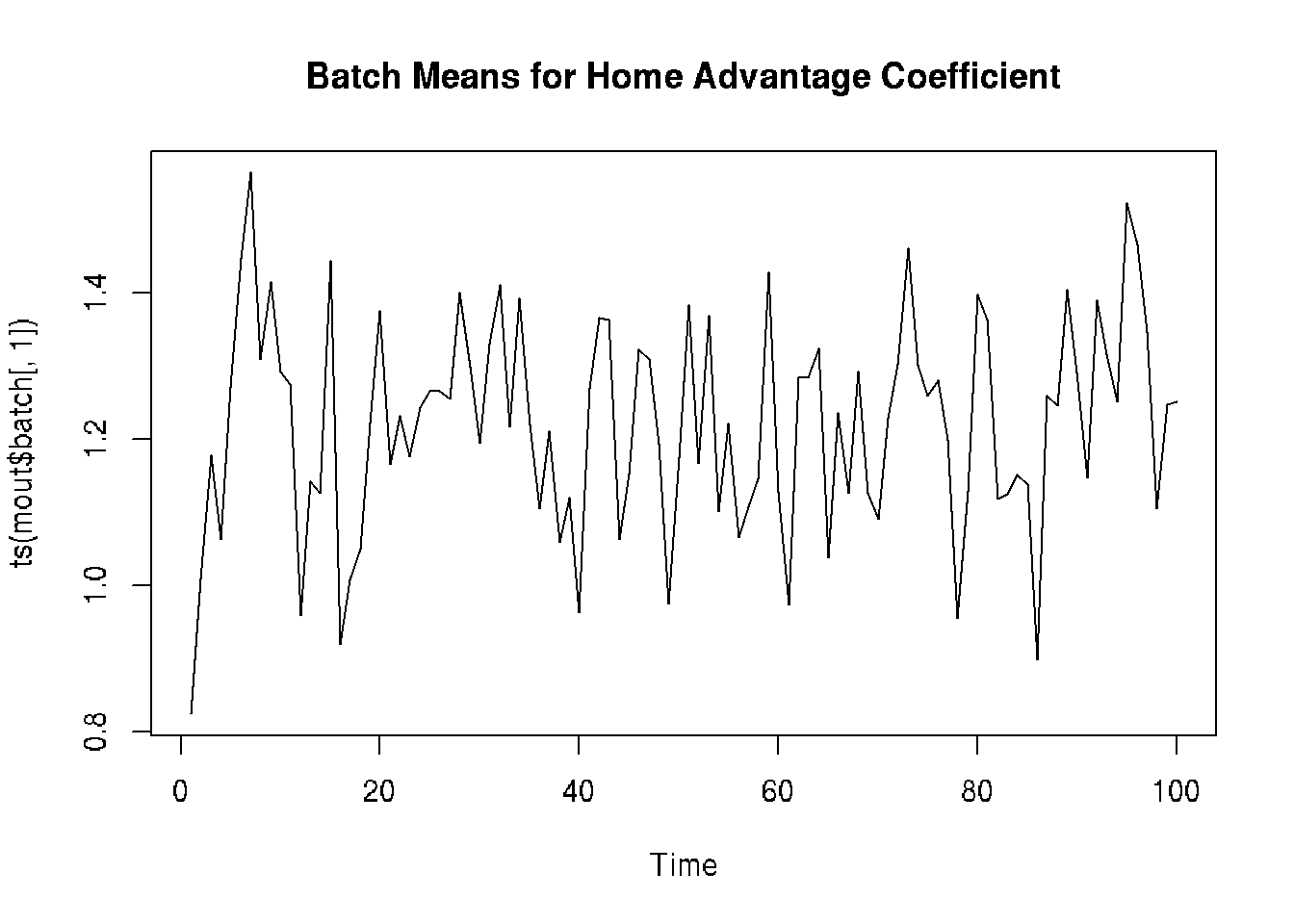plot(ts(mout$batch[ , 2]), main="Batch Means for Coefficient for Nebraska")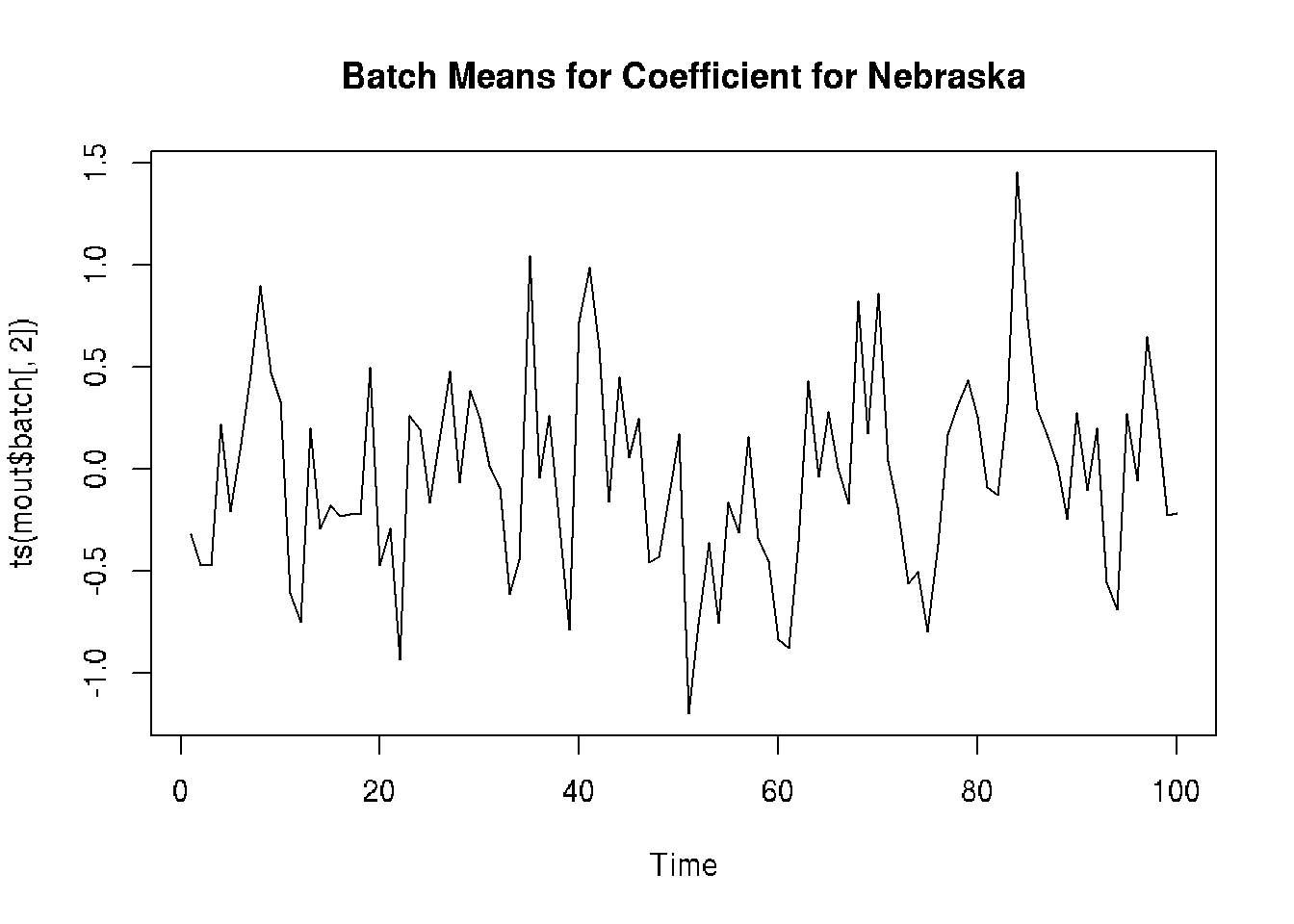plot(ts(mout$accept.batch), main="Batch Means for Acceptance Indicators")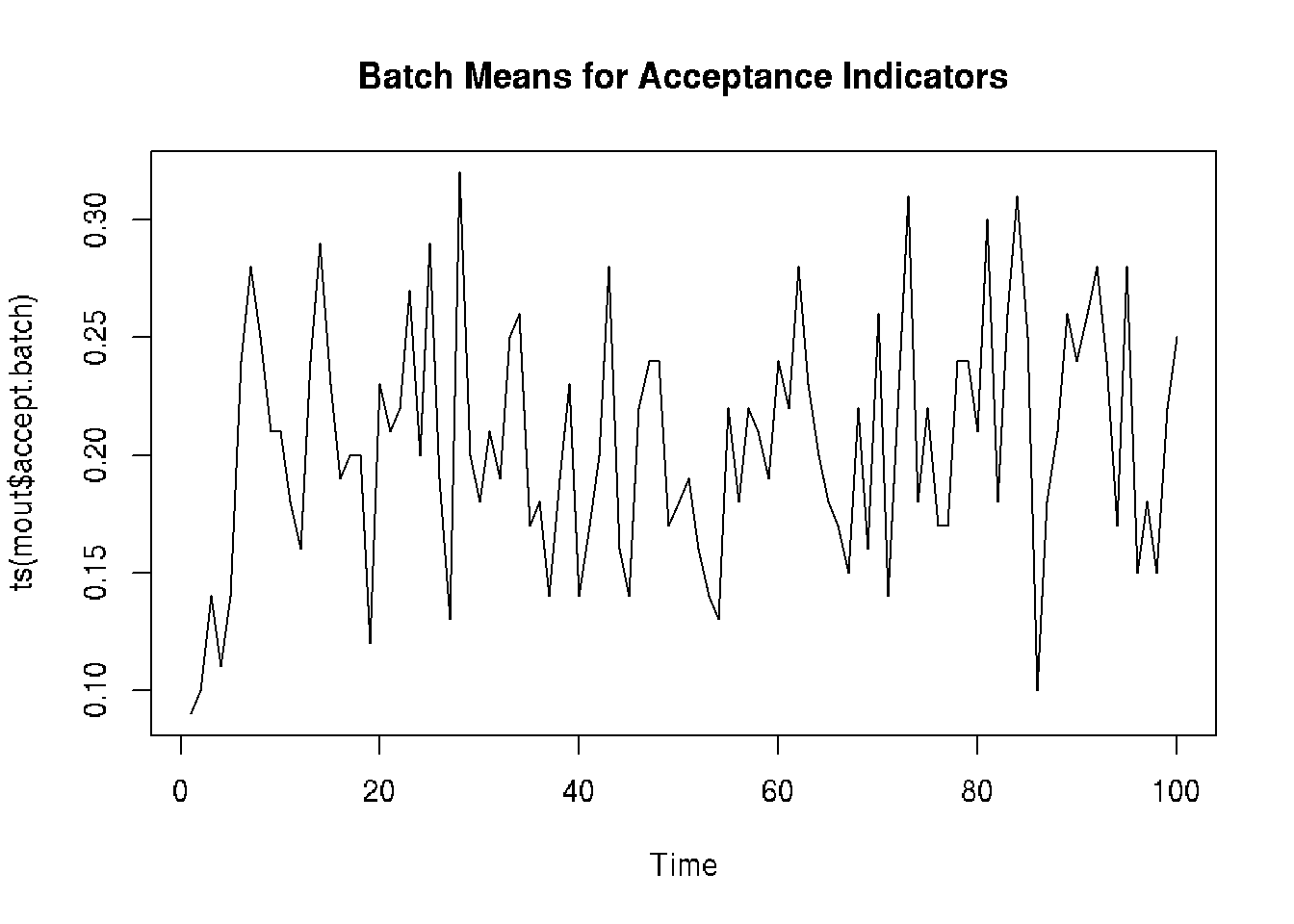##### 9.3.3.2.2 Autocorrelation Plots These give autocorrelation of the time series of batch means. Autocorrelation is correlation of the time series with itself at a later time. There should not be significantly nonzero correlation except for lag zero. Otherwise the batch length is not long enough. acf(ts(mout$batch[ , 1]), main="Autocorrelation of Home Advantage Coefficient")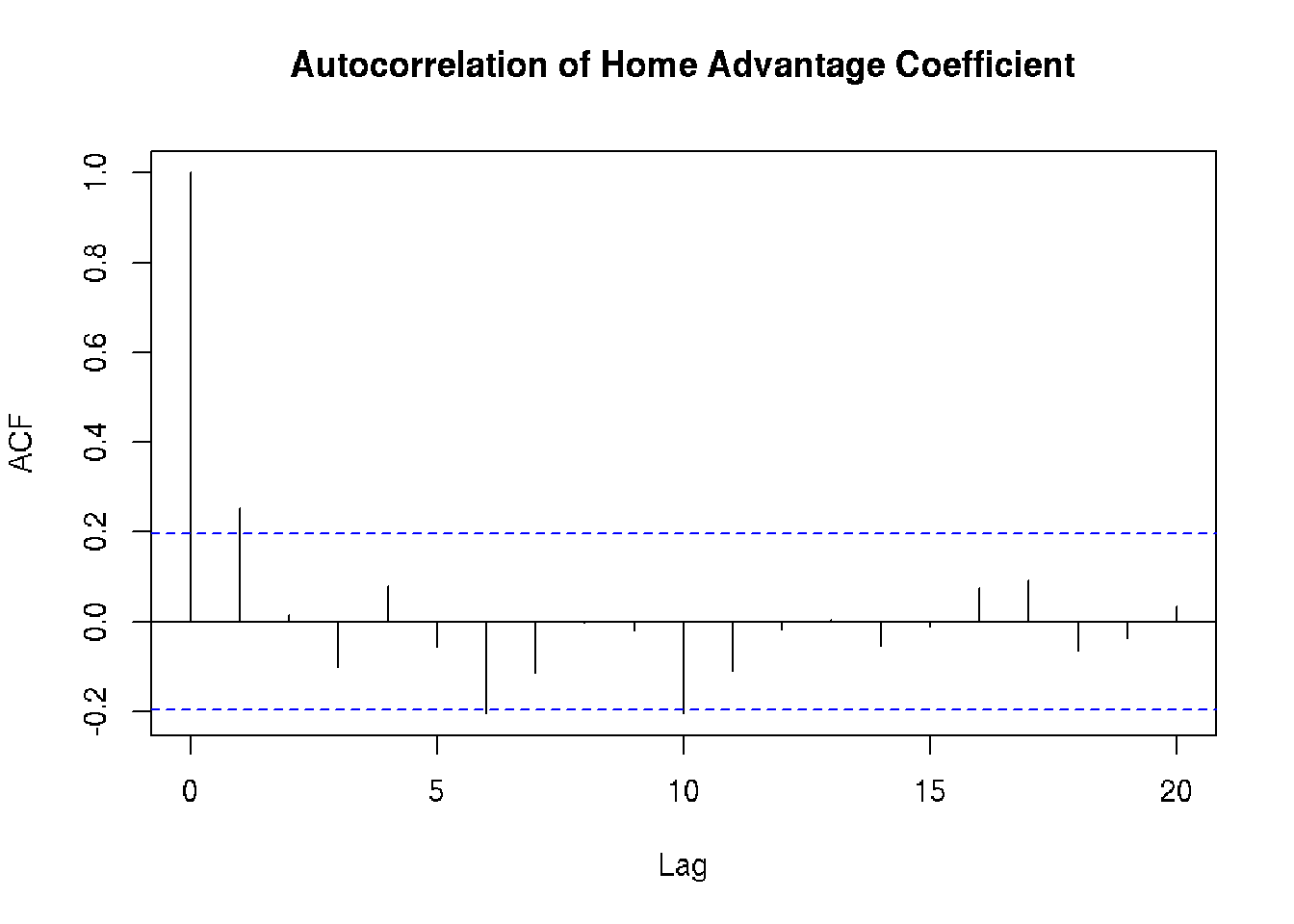acf(ts(mout$batch[ , 2]), main="Autocorrelation of Coefficient for Nebraska")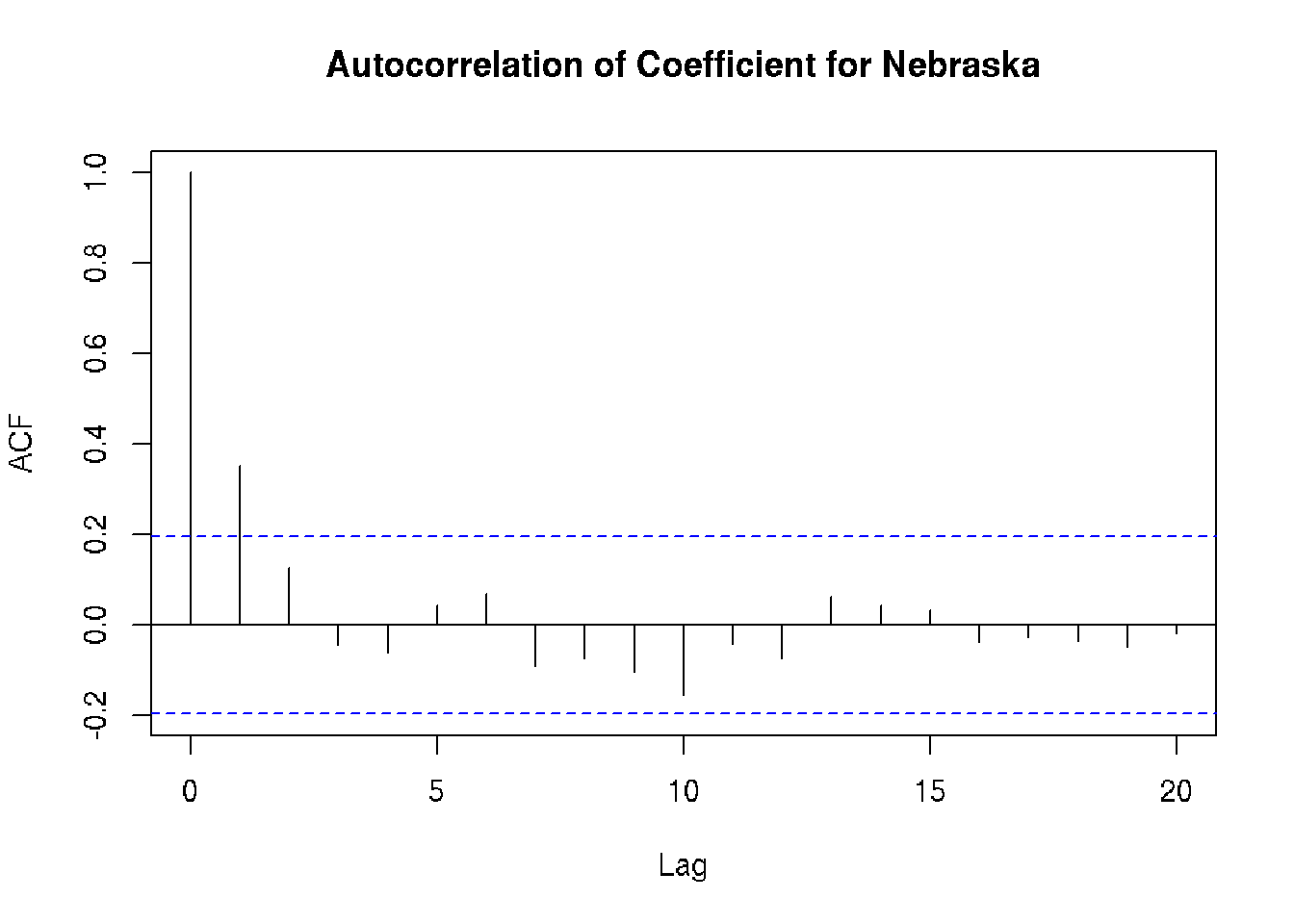acf(ts(mout$accept.batch), main="Autocorrelation of Acceptance Indicators")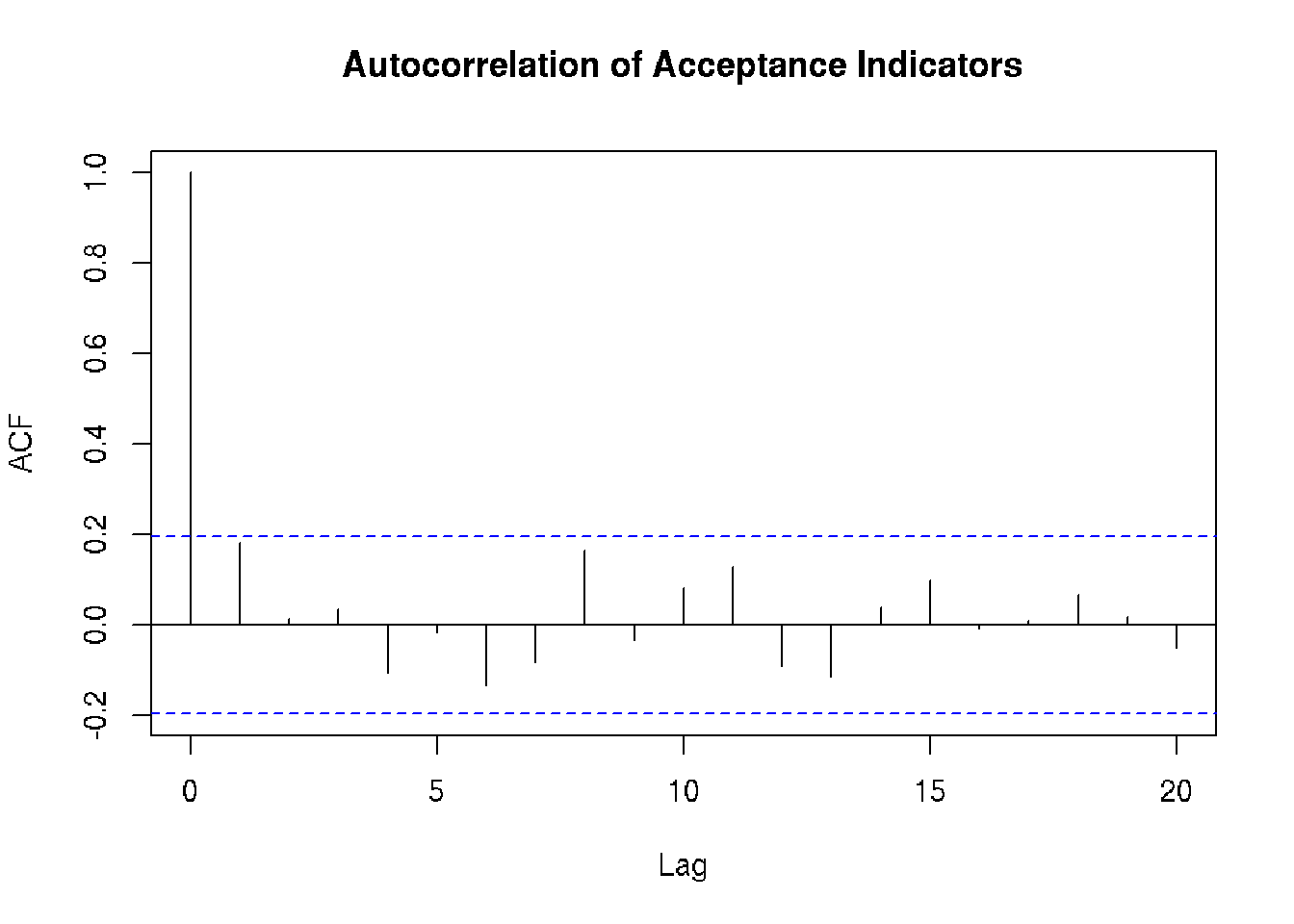It seems that at least some of the lag one autocorrelations are significant (the blue dashed lines are supposed to be cutoff points for 0.05 level tests of the null hypothesis that the true unknown autocorrelation is zero. Hence we should double the batch length (or more) to be safe.

#### 9.3.3.3 Output Function

We also want to make this example a philosophical example where the Bayesian answers questions that the frequentist cannot make sense of.

The issue we will address is which team is best, and more specifically what is the probability that each team is best, a question of about probability of parameters that the frequentist denies makes any sense (because the frequentist insists that parameters are not random).

We have applied Bayes’ rule already. We have sampled the posterior. But we have not examined the function of those parameters that addresses this question. In order to calculate the probabilities of these events, we need to output the indicators of these events.

outfun <- function(beta) {
stopifnot(is.numeric(beta))
stopifnot(is.finite(beta))
stopifnot(length(beta) == ncol(modmat))
beta <- beta[- 1] # get rid of home court advantage parameter
as.numeric(beta == max(beta))
}

#### 9.3.3.4 Try 2

mout <- metrop(mout, blen = 1000, outfun = outfun)
mout$time ## user system elapsed ## 12.724 0.012 12.760 mout$accept
##  0.19991

Starting to take a noticeable amount of computing time (12.8 sec). But clearly we could run a lot longer if we wanted more precise answers.

colnames(mout$batch) ## NULL colnames(mout$batch) <- names(gout$coefficients)[-1] foo <- as.ts(mout$batch)
colMeans(foo)
##       Illinois        Indiana           Iowa       Maryland       Michigan
##        0.00000        0.00000        0.00000        0.00000        0.00000
## Michigan.State      Minnesota       Nebraska   Northwestern     Ohio.State
##        0.00177        0.30041        0.50489        0.00000        0.00000
##     Penn.State         Purdue        Rutgers      Wisconsin
##        0.00560        0.00000        0.00000        0.18733

In a run this short we do not see any samples from the posterior in which any but the estimated top 5 in the frequentist analysis have any probability of being the actual best. A longer run might have such realizations, giving nonzero estimates for some other teams.

The theory of importance sampling Geyer, 2011, Handbook of Markov Chain Monte Carlo, Chapter 11 would allow precise estimates of the very small probabilities that our crude analysis estimates to be zero, but we won’t bother with that.

The results we have here are good enough for us. Only the estimated top 3 teams have appreciable probability of having been the actual best.

Let us limit the rest of the analysis to them

foo <- foo[ , colMeans(foo) > 0.01]
colMeans(foo)
## Minnesota  Nebraska Wisconsin
##   0.30041   0.50489   0.18733
apply(foo, 2, sd) / sqrt(mout$nbatch) ## Minnesota Nebraska Wisconsin ## 0.009313411 0.010705666 0.008035943 The last line is the Monte Carlo standard errors (MCSE) of the (Monte Carlo estimates) of the posterior probabilities which are the line above. We see that in this run taking 12.8 sec we have almost 2 significant figures (error of one or two in the second decimal place). The square root law says that to get one more significant figure (10 times the accuracy) we need to run 100 times as long (21.3 min), and we won’t bother with that here. #### 9.3.3.5 Diagnostic Plots for Try 2 plot(foo)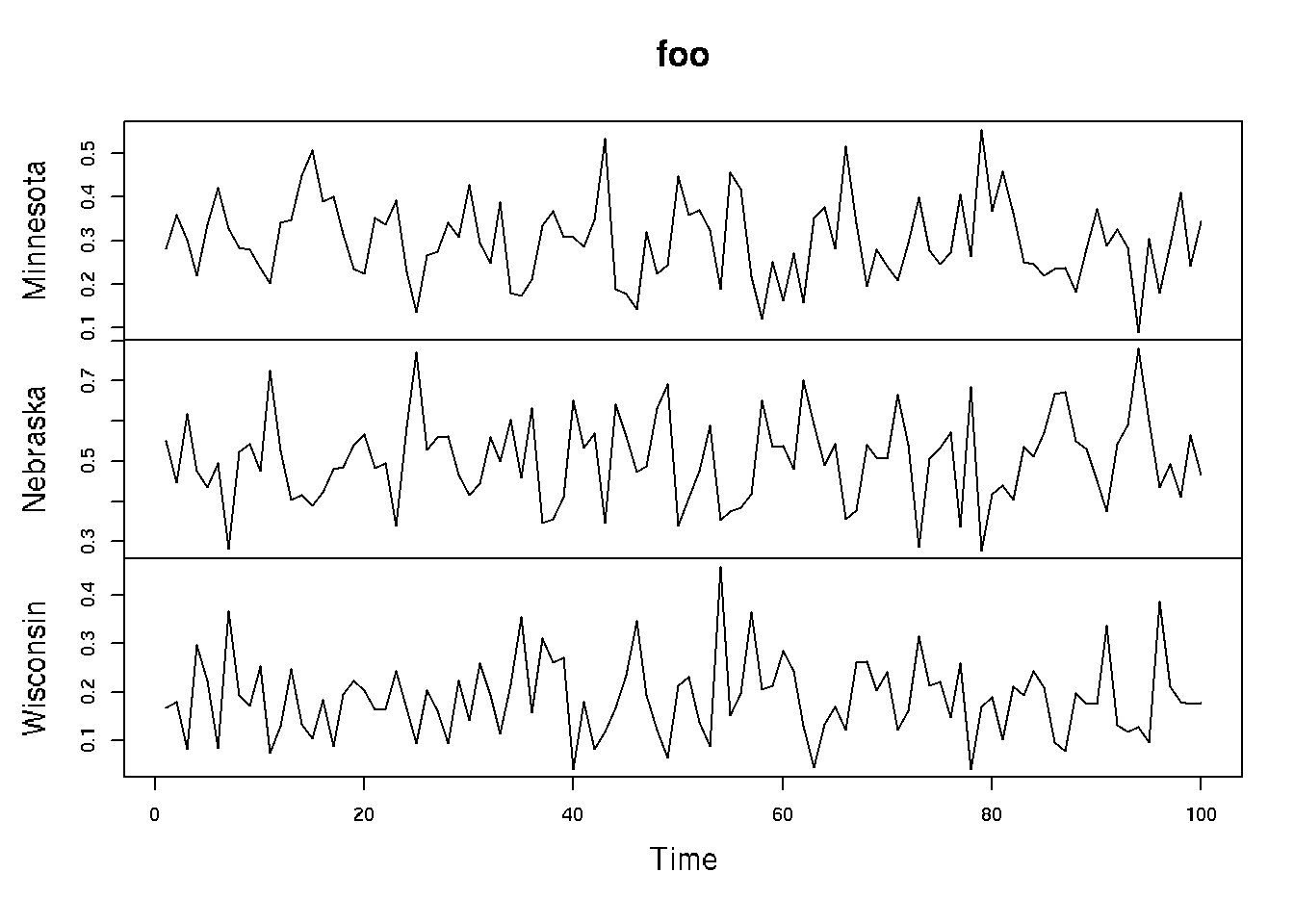acf(foo)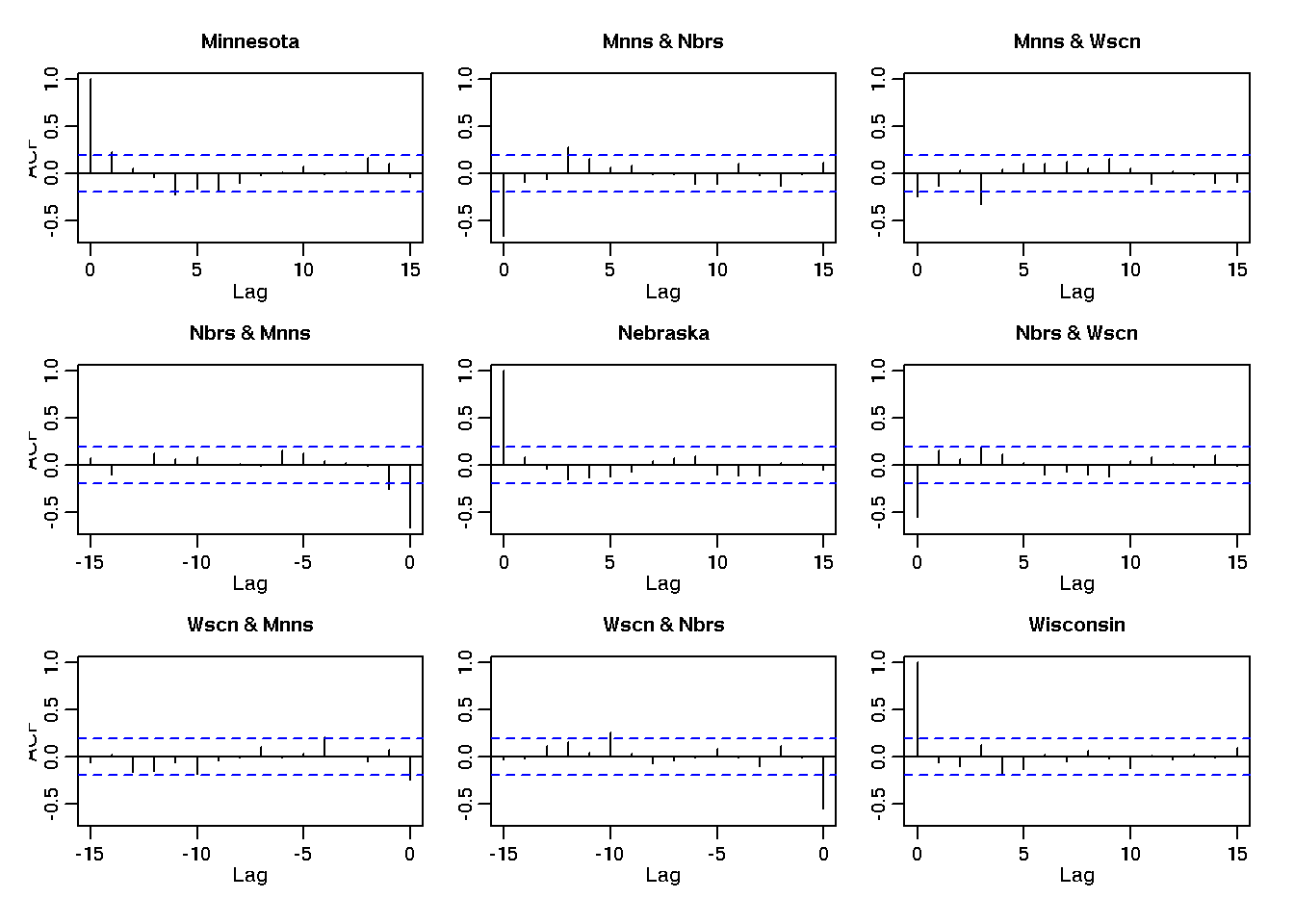These look OK with almost all of the autocorrelations and auto-cross-correlations (except for lag 0, which we are not trying to make small) are smaller than the blue lines and those that cross the blue lines only stick out a small amount). Remembering about correction for multiple hypothesis testing we ignore them. (It is very confusing that the theory of MCMC is frequentist, so our analysis of MCMC is also frequentist, even though the application we are doing is Bayesian. Have to always keep that in mind. I made a comment to this effect in my first ever public talk about MCMC at the first ever MCMC workshop for statisticians, in 1991 just as the bandwagon for Bayesian MCMC was starting to roll. During the question period a Bayesian in the audience, which was almost all Bayesians, said he was glad that I had recognized that frequentist inference was only good for analyzing Monte Carlo where the (Monte Carlo) sample size can be made as large a you please by running the computer longer. This was what I had said except for the only, which made it not at all what I meant. But it got a huge laugh from the Bayes partisans. So I just smiled and didn’t argue and went on to the next question.) Anyway, in summary, we have our results. bar <- rbind(colMeans(foo), apply(foo, 2, sd) / sqrt(mout$nbatch))
rownames(bar) <- c("probability of being best", "MCSE")
knitr::kable(bar, digits = 2, caption = "Estimated Posterior Probability of Being the Best Team in the Conference.  Probabilities to not add to one; there was small probability of other teams being the best.")
Estimated Posterior Probability of Being the Best Team in the Conference. Probabilities to not add to one; there was small probability of other teams being the best.
probability of being best 0.30 0.50 0.19
MCSE 0.01 0.01 0.01

## 9.4 Hierarchical Bayesian Analysis

### 9.4.1 Prior and Hyperprior

It is a possible problem with our first analysis that the prior seems completely arbitrary. Why does each prior for each parameter involve two games, one a win and the other a loss? Isn’t that completely arbitrary and unjustified? Yes!

One of the standard tricks to deal with objections like that is to use a parametric family of priors. It turns out that $g(\beta \mid \nu) = \frac{1}{B(\nu, \nu)} \cdot \bigl[ p(\beta) q(\beta) \bigr]^\nu, \qquad - \infty < \beta < \infty,$ where $$B$$ (which is supposed to be the Greek letter capital beta rather than the Roman letter capital b, but they are not distinguished typographically) is a special function (the beta function) that R knows about (R function beta), is a normalized probability distribution for any $$\nu > -1$$ and also that $g(\nu) = e^{- \nu}, \qquad 0 < \nu < \infty$ is also a normalizable probability distribution. We are going to use these for our priors.

We are not going to delve into the theoretical details why these are facts. When you take the theory course, you will learn that the first is derived from the beta distribution using the change-of-variable theorem and the second is the exponential distribution.

The story is that when we use this conditional $$g(\beta \mid \nu)$$ as a prior for (some) $$\beta$$, then we have another parameter $$\nu$$ in this prior. This gets confusing when the distribution for the parameter has a parameter. What is “parameter” supposed to refer to?

So the jargon calls $$\beta$$ a parameter (as we did before) and $$\nu$$ a hyperparameter. Then any parameters in the distribution of $$\nu$$ (mercifully, in this example there aren’t any) would be called hyperhyperparmeters and so forth. And the prior for the hyperparameter is called a hyperprior. This pile of priors, hyperpriors, hyperhyperpriors, and so forth is called “hierarchical Bayes” in the jargon.

It is important to understand that hierarchical Bayes is not some sort of generalization of ordinary Bayes. It is a special case of ordinary Bayes. There is no “hyper” in probability theory, so there is no “hyper” in Bayes rule. All of the parameters, hyperparameters, hyperhyperparameters, and however many “hypers” one wants to throw in there, are just parameters (a rose by any other name would smell_as sweet). The pile of prior, hyperprior, hyperhyperprior, and however many “hypers” one wants to throw in there, multiply together to make the joint prior distribution of all the parameters. So there is no mathematical or statistical content in the hierarchical Bayes story. It is just a kind of blather that certain Bayesians think helps organize their thinking.

The woof is that we are using a more flexible family of priors for $$\beta$$ that includes what we had before (the case $$\nu = 1$$) as a special case. And then we are letting the data choose what $$\nu$$ is right! Of course, the posterior distribution of $$\nu$$ still depends on our prior for $$\nu$$.

Also we didn’t even have $$\nu$$ in the problem to begin with. It is completely a theoretical artifact — not a parameter of the distribution of the data.

Another way to think of what we are doing that ignores the woof about hierarchicality is to say that we are just using a different prior for $$\beta$$, whatever we get when we integrate out $$\nu$$. But we don’t know how to do that integral. So that means we don’t actually know what prior we are using for $$\beta$$ (marginally). More on this below.

Also $$\beta$$ is a vector and if we use the same $$\nu$$ for the different $$\beta$$’s, then we get a prior that makes the components of the coefficient vector correlated. And that means we understand what we are doing even less.

Nevertheless, hierarchical Bayes schemes are very widely used and we shall follow the herd in this respect (despite our misgivings).

Wanting to treat the teams evenhandedly suggests that we want to use the same $$\nu$$ for all the team coefficients. But since the home court advantage coefficient is fundamentally different, it can have a different $$\nu$$. That gives us a prior, in the notation above, where we call the home court advantage coefficient $$\gamma$$ and the rest $$\beta_i$$, $$i = 1$$, $$\ldots,$$ $$p$$, our prior is $\left[ \frac{1}{B(\nu_1, \nu_1)^p} \prod_{i = 1}^{p} p(\beta_i)^{\nu_1} q(\beta_i)^{\nu_1} \right] \frac{1}{B(\nu_2, \nu_2)} p(\gamma)^{\nu_2} q(\gamma)^{\nu_2} \cdot e^{- \nu_1} e^{- \nu_2}$

### 9.4.2 Log Prior and Log Posterior

And the log prior is $- p \log B(\nu_1, \nu_1) - \log B(\nu_2, \nu_2) - \nu_1 - \nu_2 - \nu_2 \bigl[ \log p(\gamma) + \log q(\gamma) \bigr] - \nu_1 \sum_{i = 1}^p \bigl[ \log p(\beta_i) + \log q(\beta_i) \bigr]$

So as an R function it is

# decide where in the state vector the various parameters will be
idx.gamma <- 1
idx.beta <- seq(2:ncol(modmat))
idx.nu1 <- ncol(modmat) + 1
idx.nu2 <- ncol(modmat) + 2
# also make some helper functions
logp <- function(eta) ifelse(eta < 0, eta - log1p(exp(eta)),
- log1p(exp(- eta)))
logq <- function(eta) ifelse(eta < 0, - log1p(exp(eta)),
- eta - log1p(exp(- eta)))

log.prior <- function(theta) {
stopifnot(is.numeric(theta))
stopifnot(is.finite(theta))
stopifnot(length(theta) == ncol(modmat) + 2)
gamma <- theta[idx.gamma]
beta <- theta[idx.beta]
nu1 <- theta[idx.nu1]
nu2 <- theta[idx.nu2]
if (nu1 <= 0 || nu2 <= 0) return(-Inf)
- length(beta) * lbeta(nu1, nu1) - lbeta(nu2, nu2) - nu1 - nu2 -
nu2 * (logp(gamma) + logq(gamma)) -
nu1 * sum(logp(beta) + logq(beta))
}
log.unnormalized.posterior <- function(theta) {
stopifnot(is.numeric(theta))
stopifnot(is.finite(theta))
stopifnot(length(theta) == ncol(modmat) + 2)
# beta in this function is the argument for logl
# all the regression coefficients, including home court advantage
beta <- theta[1:ncol(modmat)]
logl(beta) + log.prior(theta)
}

### 9.4.3 Try 1

Now we need a starting state that has positive probability, so any values for the regression coefficients and positive values for $$\nu_1$$ and $$\nu_2$$. Before we were assuming $$\nu_1 = \nu_2 = 1$$ and we have a probable value of the regression coefficients for that (where the last run of MCMC stopped). So use that.

theta.start <- c(mout$final, 1, 1) And we are ready to start over, doing MCMC on this new Bayesian model (same likelihood, different prior, including hyperparameters). We use the same scale we did before hoping it still works. mout <- metrop(log.unnormalized.posterior, theta.start, nbatch = 100, blen = 100, scale = 0.4) mout$accept
##  0.5025
mout <- metrop(mout, scale = 0.5)
mout$accept ##  0.4937 ### 9.4.4 Debug Try 1 That’s ugly. Changing the scale should have changed the acceptance rate. What is going on? plot(mout$batch[ , ncol(modmat) + 1], ylab = "nu1")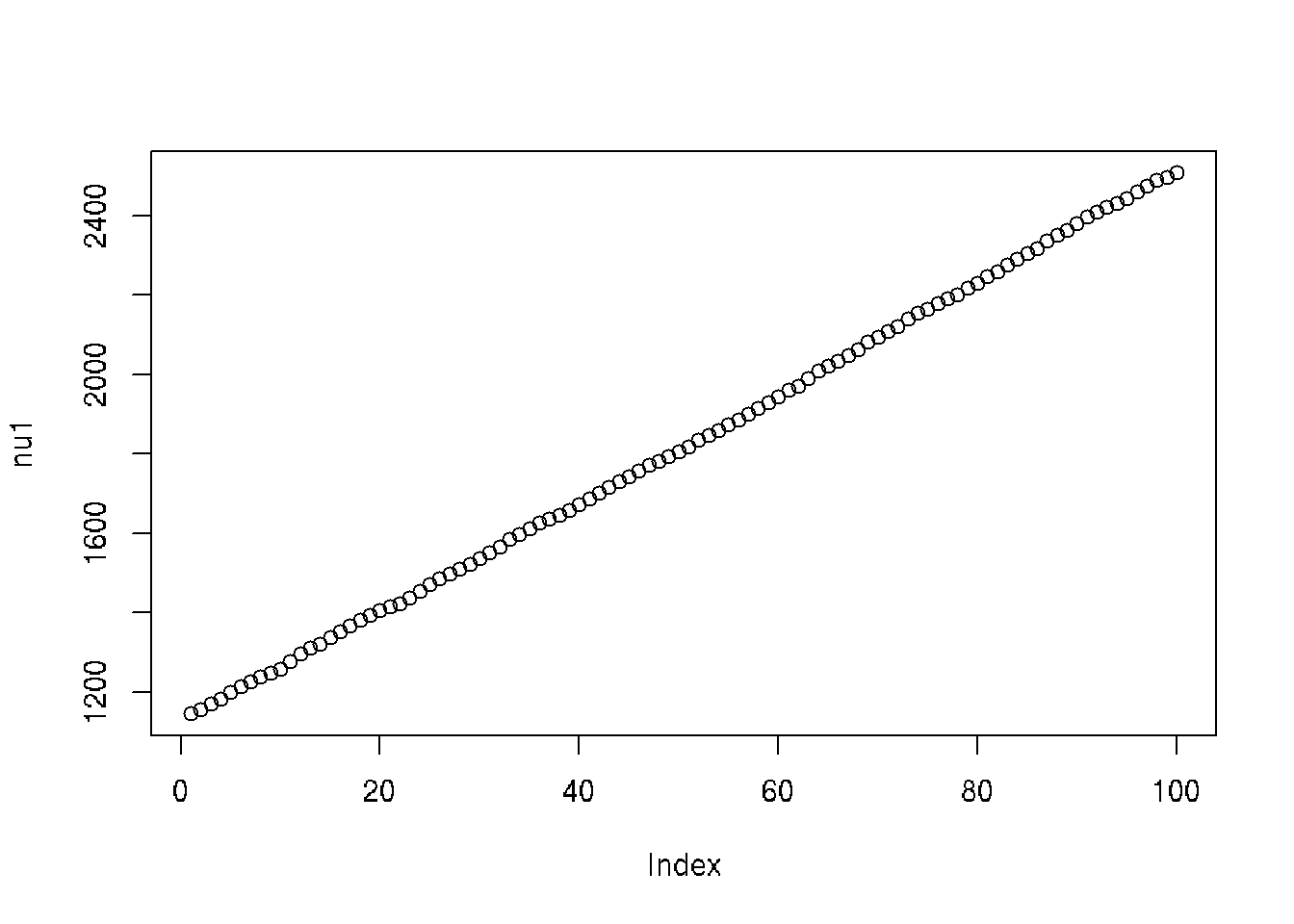plot(mout$batch[ , ncol(modmat) + 2], ylab = "nu2")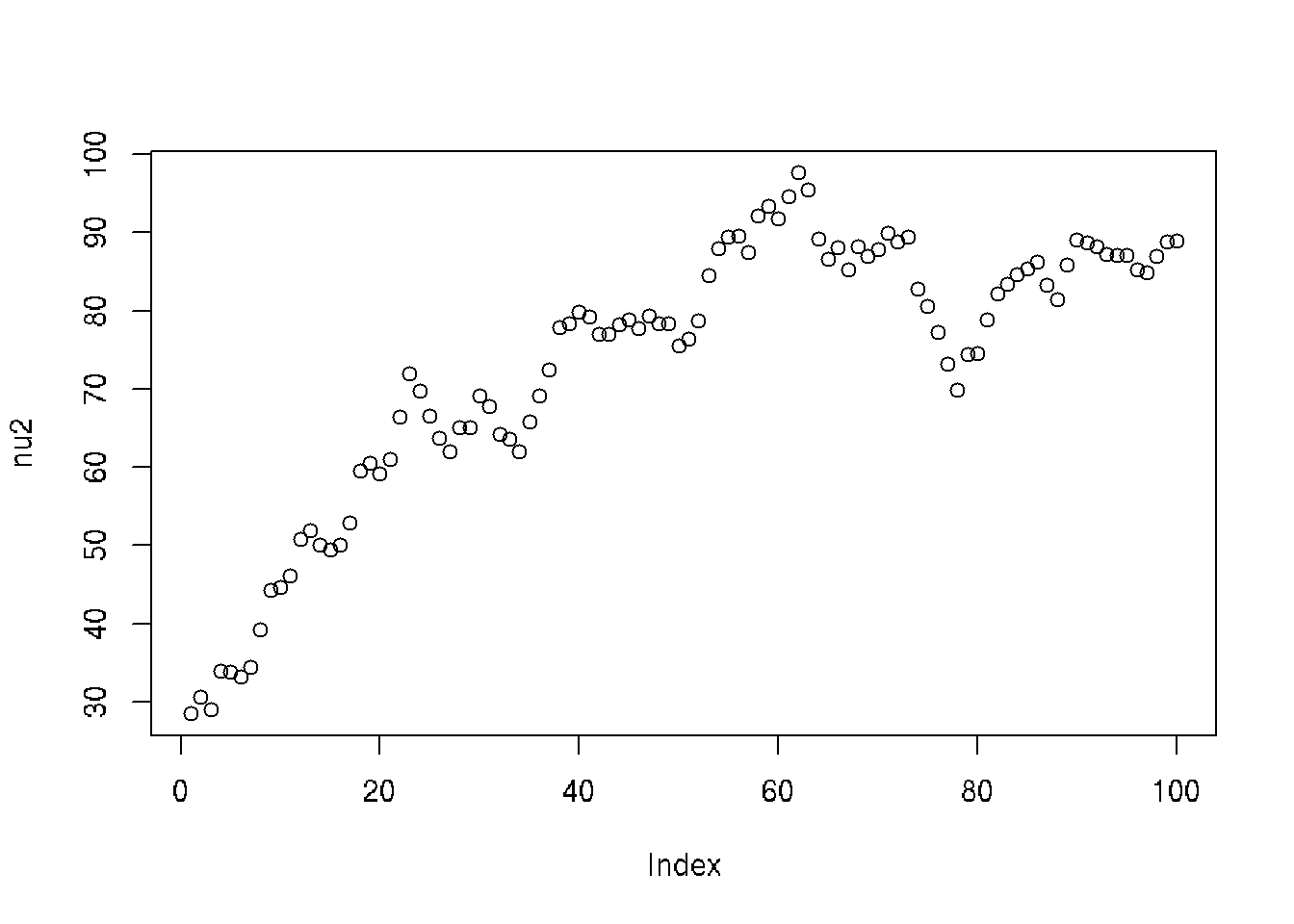Oops! Staring at the code was no help, but evaluating some of the terms in the log posterior eventually showed my sign mistake. $- p \log B(\nu_1, \nu_1) - \log B(\nu_2, \nu_2) - \nu_1 - \nu_2 + \nu_2 \bigl[ \log p(\gamma) + \log q(\gamma) \bigr] + \nu_1 \sum_{i = 1}^p \bigl[ \log p(\beta_i) + \log q(\beta_i) \bigr]$ (the last 2 terms have plus signs in front of them, not minus signs like they did before) log.prior <- function(theta) { stopifnot(is.numeric(theta)) stopifnot(is.finite(theta)) stopifnot(length(theta) == ncol(modmat) + 2) gamma <- theta[idx.gamma] beta <- theta[idx.beta] nu1 <- theta[idx.nu1] nu2 <- theta[idx.nu2] if (nu1 <= 0 || nu2 <= 0) return(-Inf) - length(beta) * lbeta(nu1, nu1) - lbeta(nu2, nu2) - nu1 - nu2 + nu2 * (logp(gamma) + logq(gamma)) + nu1 * sum(logp(beta) + logq(beta)) } ### 9.4.5 Try 2 Start over. mout <- metrop(log.unnormalized.posterior, theta.start, nbatch = 100, blen = 100, scale = 0.4) mout$accept
##  0.13
mout <- metrop(mout, scale = 0.3)
mout$accept ##  0.2364 #### 9.4.5.1 Diagnostic Plots In any hierarchical Bayes scheme you expect the highest level of hyper, hyper-hyper, hyper-hyper-hyper, or whatever, to be the slowest mixing (most autocorrelation) because it is farthest from the observed data. plot(as.ts(mout$batch[ , ncol(modmat) + 1]), ylab = "nu1")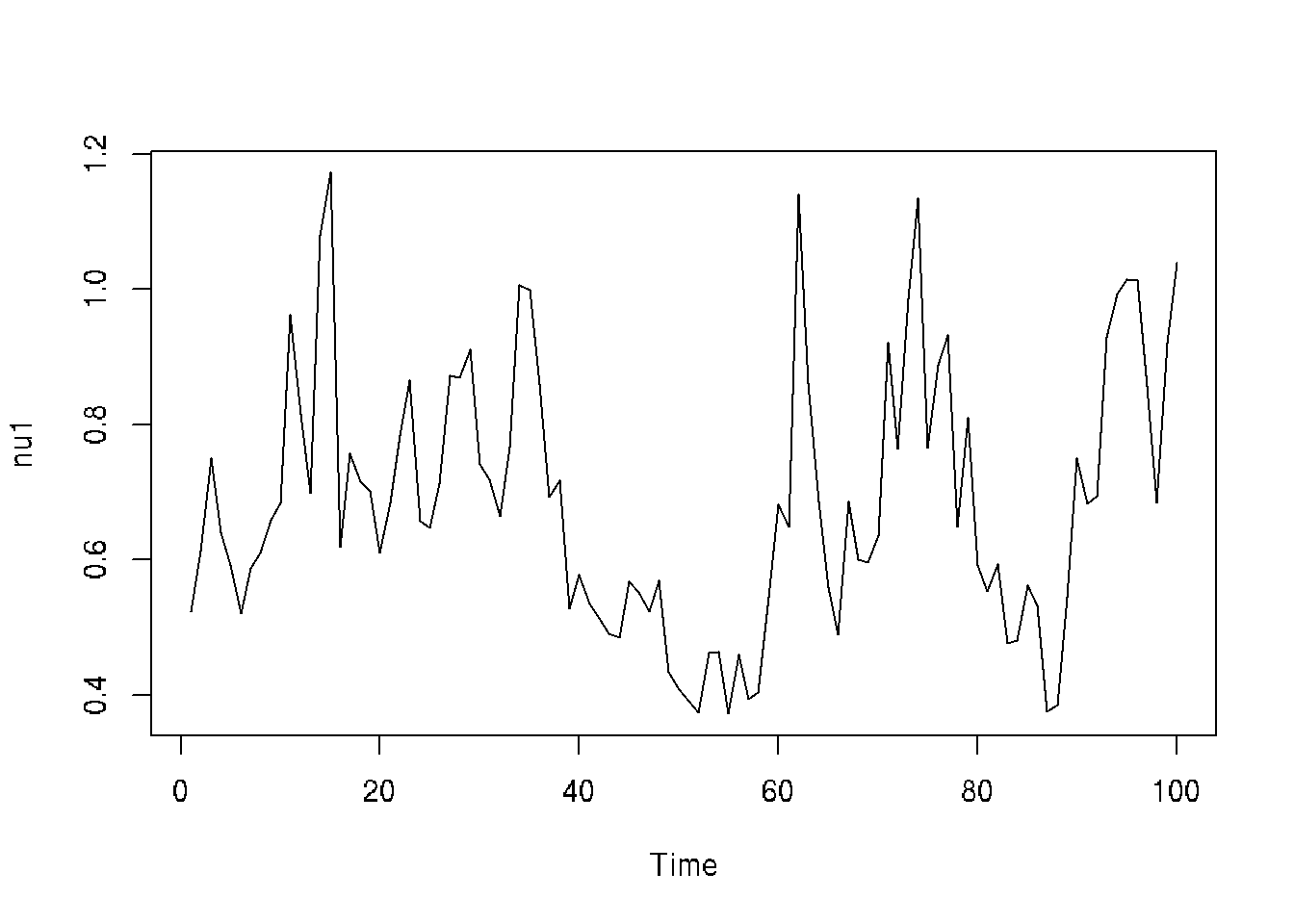plot(as.ts(mout$batch[ , ncol(modmat) + 2]), ylab = "nu2")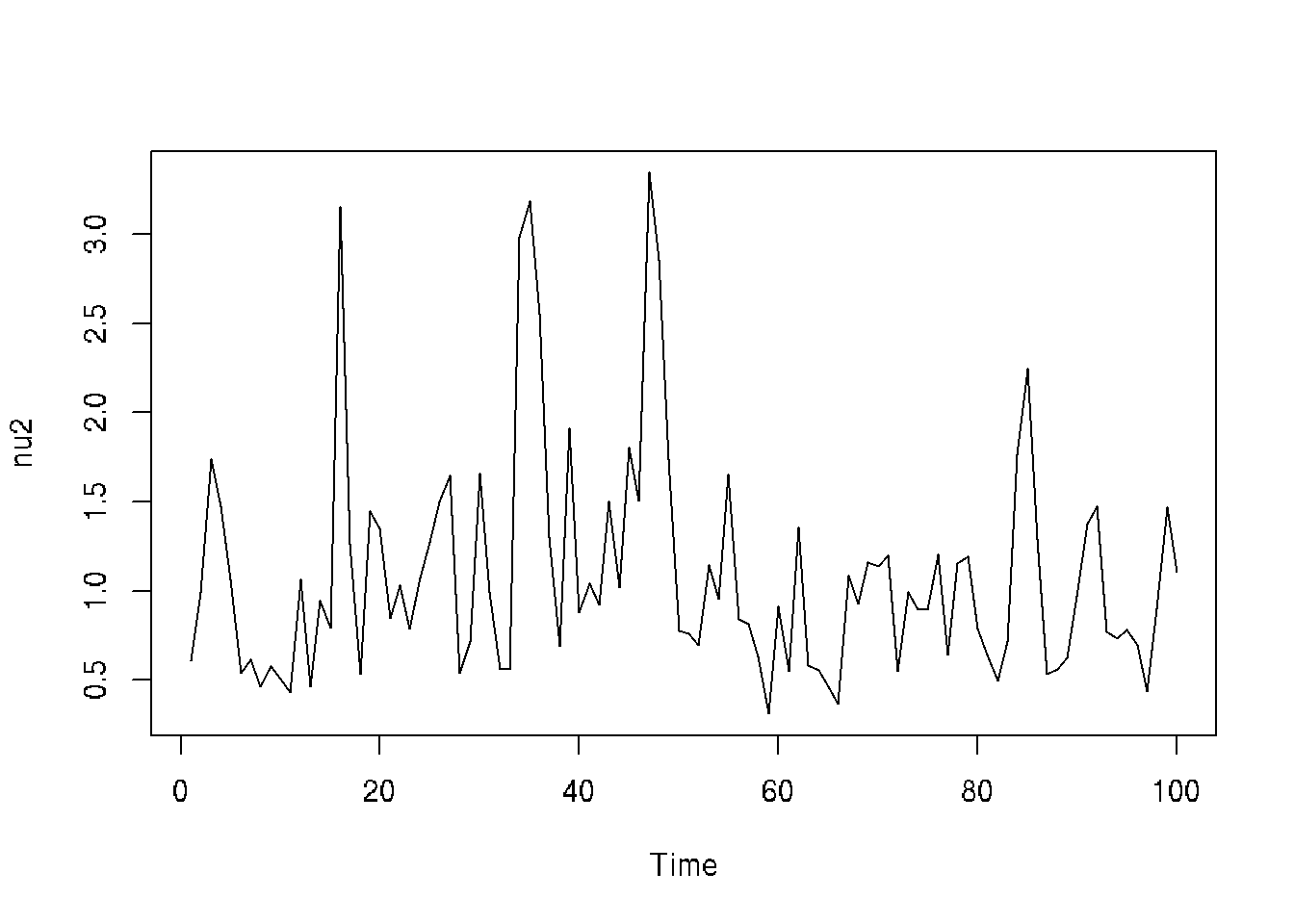acf(mout$batch[ , ncol(modmat) + 1], main = "Series nu1")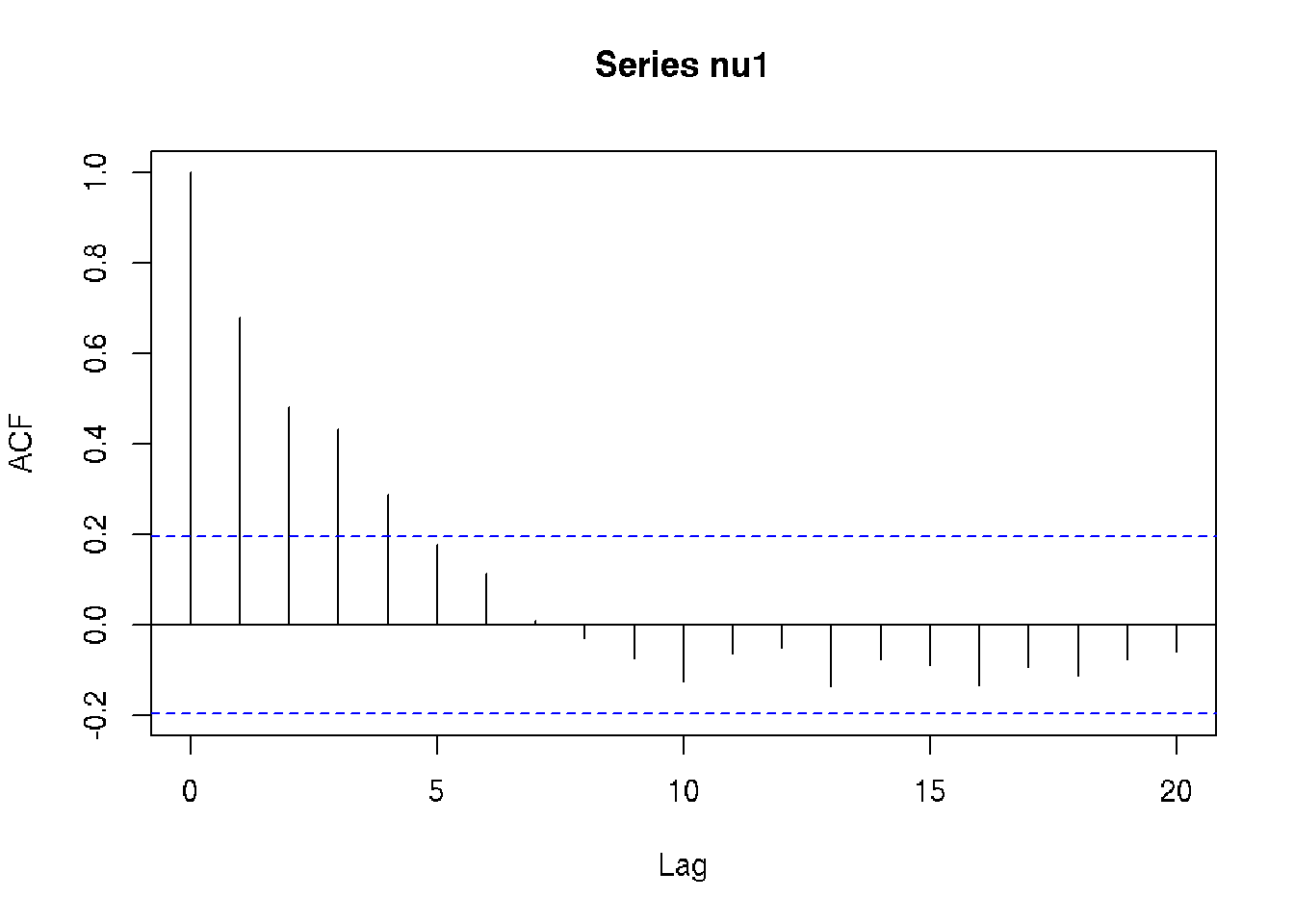acf(mout$batch[ , ncol(modmat) + 2], main = "Series nu2")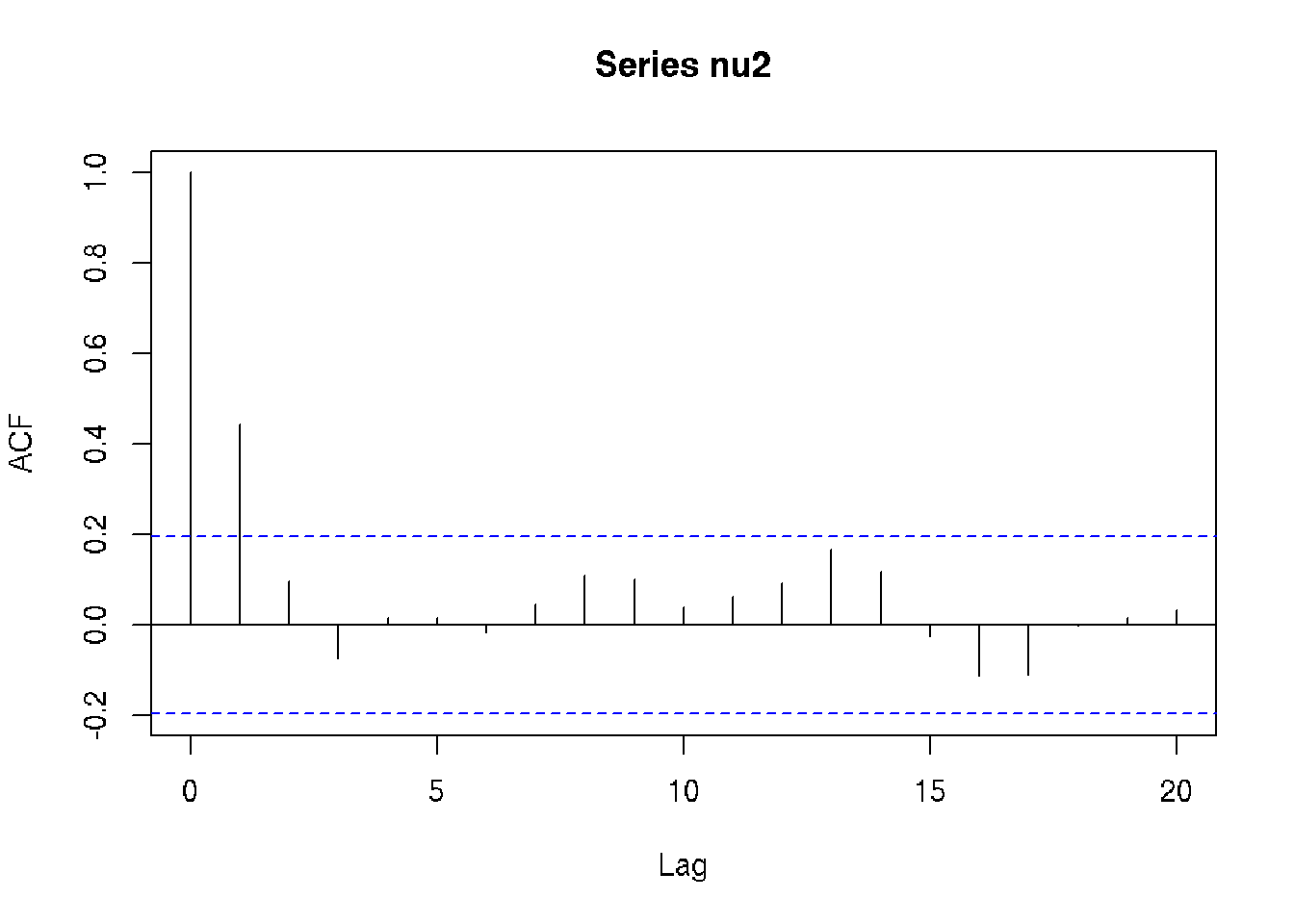It is clear that this model mixes a lot slower than the first model. We need at least 5 times the batch length to use the method of batch means. But blen = 1000 like we had before for our final run should be safe ### 9.4.6 Try 3 But rather than repeat the analysis we did with our first model, we look at some different questions. What are the (marginal) posterior distributions of these hyperparameters? We need samples of those two parameters unbatched because density estimation isn’t about averaging. So we need a run with no batching. But we don’t really need zillions of samples. So we space the samples instead, throwing away all but every tenth one to cut down the size of the data. mout <- metrop(mout, blen = 1, nspac = 10, nbatch = 1e5, outfun = c(idx.nu1, idx.nu2)) mout$accept
##  0.235673

When outfun is a numeric or logical vector rather than a function it means select those components of the state vector.

dim(mout$batch) ##  100000 2 nu1 <- mout$batch[ , 1]
nu2 <- mout\$batch[ , 2]
acf(nu1, lag.max = 300)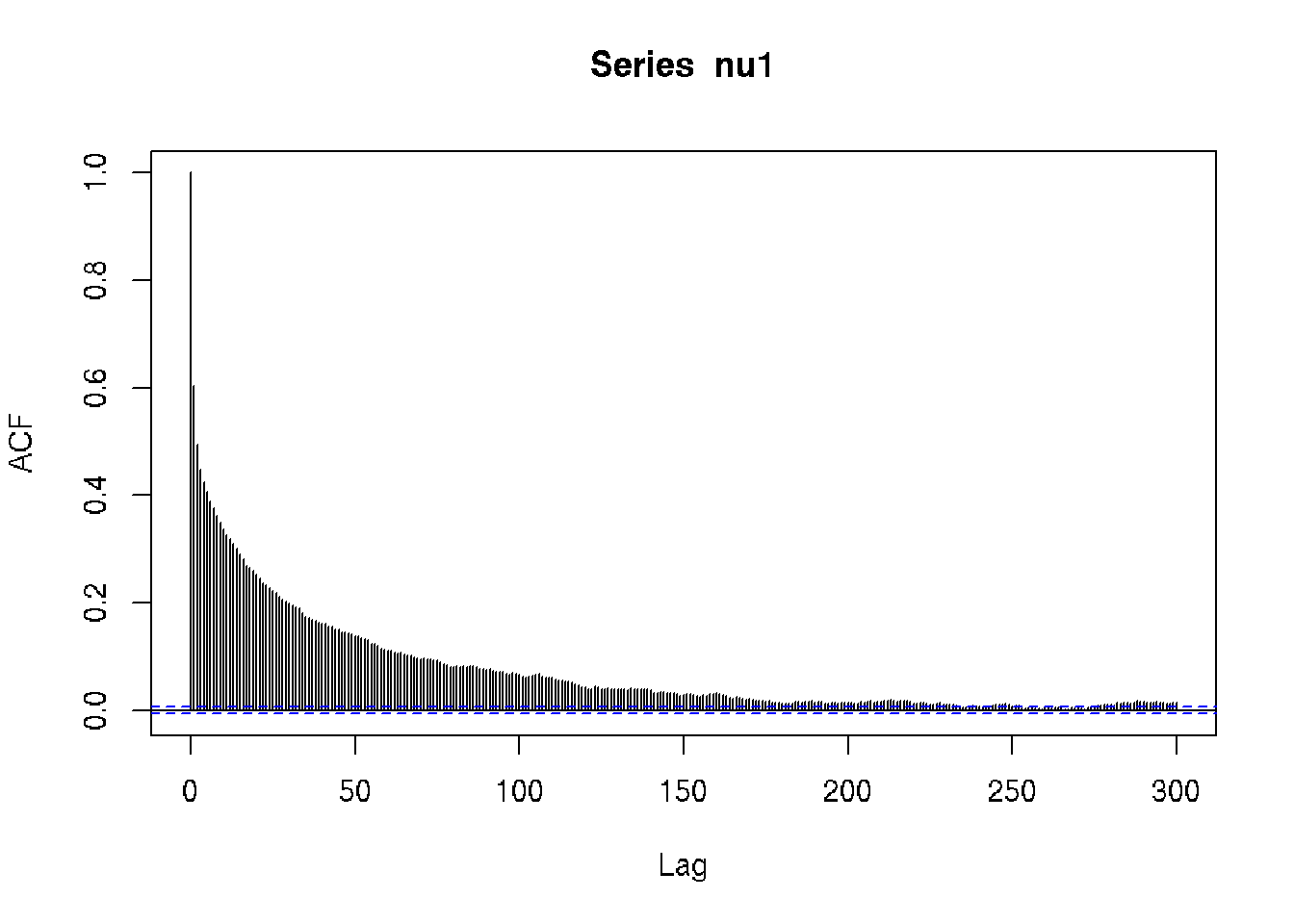acf(nu2, lag.max = 100)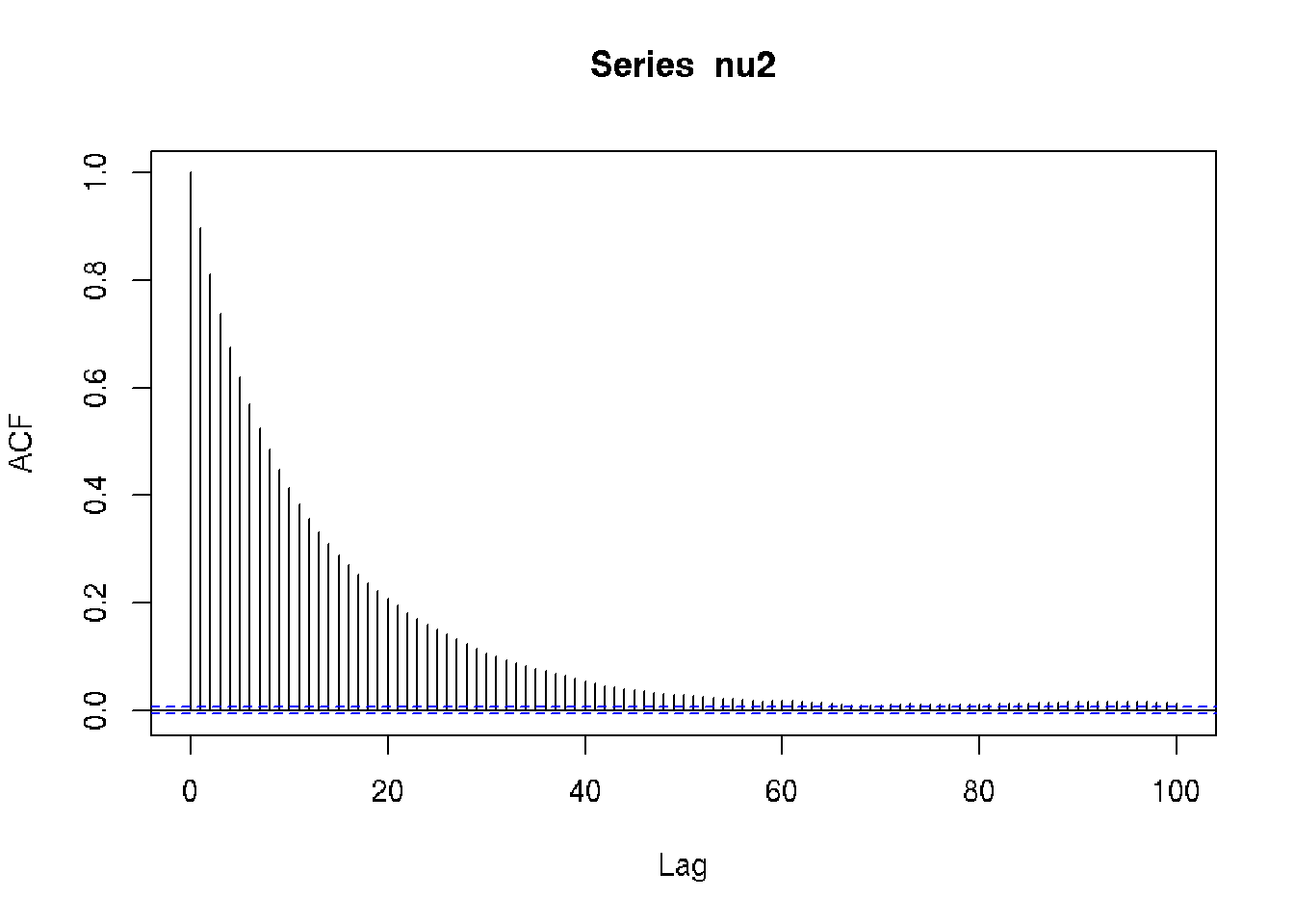We want to do density estimation with automatic “bandwidth” selection as we did in Section 5.4 of the course notes on simulation, but there is a problem.

Standard density estimation software expects IID data. Its bandwidth selection depends on that and will be incorrect for non-IID data. We cannot get exact independence in Markov chain samples. But we can at least get almost uncorrelated by subsampling further.

idx.sub <- seq(1, length(nu1), by = 500)
nu1.sub <- nu1[idx.sub]
nu2.sub <- nu2[idx.sub]
acf(nu1.sub)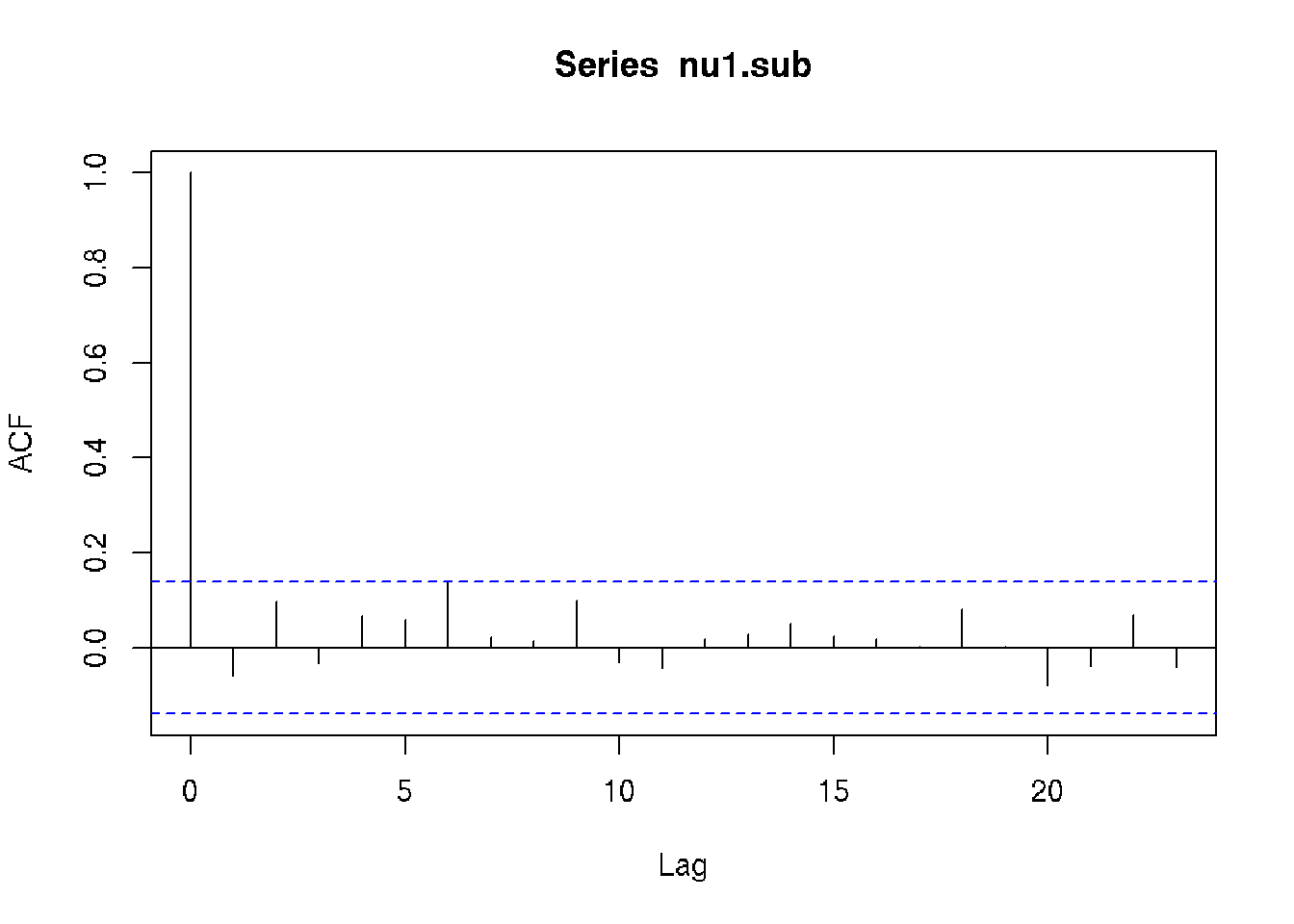acf(nu2.sub)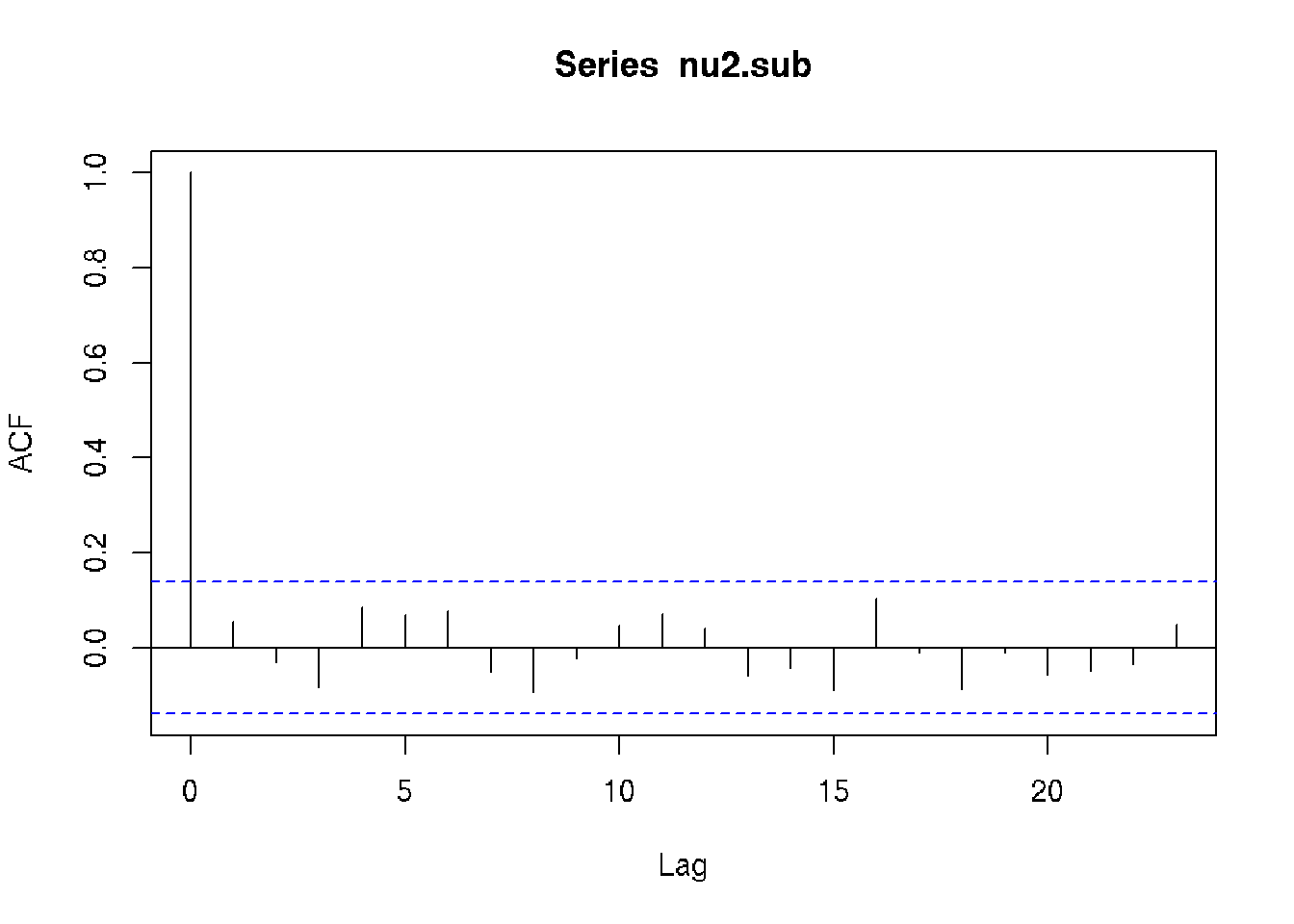So we use nu1.sub and nu2.sub to determine bandwidth and then use nu1 and nu2 for the density estimation.

bw <- dpik(nu1.sub)
den <- bkde(nu1, bandwidth = bw)
plot(den, type = "l", xlab = expression(nu), ylab = "probability density")